# THE EQUIVALENT CIRCUIT IMITATING WAVE SCATTERING BY ARRAYS OF SPLIT-RING RESONATORS

On 20 January 2019, the theoretical physicist Prof. Algirdas Matulis celebrated his 80th birthday. His paper following this text is written on this occasion.

Algirdas Matulis has graduated from Moscow University in 1962. After graduation, he returned to Vilnius and started his scientific career at the Institute of Physics and Mathematics of the Academy of Sciences of Lithuanian SSR in the group of academician A. Jucys. In 1968 he defended the thesis of a candidate of physical-mathematical sciences on the theory of an atom. Afterward, he started to work in the group of Prof. Y. Levinson at the Institute of Semiconductor Physics of the Academy of Sciences. There A. Matulis switched from the theory of an atom to the solid-state physics and defended the doctoral thesis in 1982. In 1990 he was granted the pedagogical rank of professor in physics. During 1994–1999, he was an expert member of the Lithuanian Academy of Sciences. In 1983 he was awarded the Lithuanian Science Prize, and in 1994 won the Adolfas Jucys Award, established by the Lithuanian Academy of Sciences, for his achievements in theoretical physics.

Algirdas Matulis worked as a visiting professor at Antwerp, Trondheim and Lund Universities, and Weizmann Institute. He is a co-author of more than 140 scientific papers and an author of 8 books, mostly textbooks in various fields of physics, based on the lectures he gave in Kaunas Vytautas Magnus and Vilnius Universities. His seminars on most interesting problems of theoretical physics usually collect huge audiences due to the elegant style and clarity of his presentations.

The Editorial Board of Lithuanian Journal of Physics congratulates Prof. Matulis and wishes him long fruitful years in physics.

A. Matulis, G. Šlekas, D. Seliuta, and Ž. Kancleris

Semiconductor Physics Institute, Center for Physical Sciences and Technology, Saulėtekio 3, 10222 Vilnius, Lithuania
Email: algirdas.matulis@ftmc.lt

Received 7 March 2019; accepted 2 April 2019

The scattering of waves by a periodic chain of series resonant LC circuits in a two-dimensional lattice of lumped elements is considered, that imitates the scattering of electromagnetic waves by a periodic lattice of split-ring resonators. Using the translation symmetry of this chain we transformed the scattering problem into the problem of wave propagation along the strip with a single LC circuit. This problem was solved analytically presenting the transmittance as a sum of partial transmittances, corresponding to the frequency mini-bands of the strip. It is shown that the transmittance as a function of the incident wave frequency demonstrates two types of resonances with different resonant frequency dependence on the distance between neighbouring LC circuits.

Keywords: wave scattering, split-ring resonator, 2D model of lumped elements

## 1. Introduction

The metamaterial concept has enriched optics and photonics with new and fascinating phenomena, e.g. magnetism at optical frequencies , negative index materials , electromagnetic cloaking  and strong chirality . Nowadays planar metamaterials or metasurfaces became very popular. Usually they are manufactured from resonant metallic structures, for instance, split-ring resonators (SRRs) as fundamental building blocks , formed as a periodic array on the surface of a dielectric or a semiconducting plate. Choosing suitable frequency and polarization of the incident wave, resonant oscillating currents can be excited in SRRs, resulting in transmission minima in the far-field transmission spectrum . Two types of modes can be excited in the metasurface based on SRRs by external electromagnetic wave. They are the so-called plasmonic modes, the specific frequency of which mainly depends on individual characteristics of the SRR and lattice modes that depend on the gap between SRRs in the periodic array [7, 8]. The latter can be attributed to Wood’s anomaly for the first time being noticed on metallic optical gratings that demonstrated a considerable intensity diffracted beam at nearly 90 degrees for the light normal incidence on the grating . It is worth mentioning that the lattice modes influenced the plasmonic modes and vice versa. This mutual coupling provides an additional degree of freedom for manipulating the metamaterial response: the red shift or blue shift of plasmonic modes depending on configuration of the near field , the variation in the plasmonic mode resonance depth and the resonant Q factor as a result of a change in the period or orientation of SRRs [11, 12], the enhancement of plasmonic modes in dense SRR arrays . In addition to a short-range interaction, a long-range radiative coupling at larger separations between SRRs via scattered far fields was also reported . To describe the aforementioned features of transmission spectra, the scattering problem by a periodic structure of SRRs is formulated, and Maxwell’s equations are solved numerically. Usually calculated positions of the resonances and their depth well coincide with measurement results [1, 12, 13], allowing to predict peculiarities of the periodic structures interacting with electromagnetic radiation. Due to mutual interaction of plasmon and lattice modes such models do not help much to distinguish between them . The equivalent circuit approach was also used to describe the features of a single SRR as well as of their arrays. The distributed elements were used to design the equivalent circuit in the case of an outer SRR with an inner solid ring . In the case of SRRs arrays, the reciprocal inductance between the neighbouring resonators was usually introduced to describe their mutual interaction . The equivalent circuit approach used up to now was concentrated on the elucidation of the influence of SRR array periodicity on the resonance frequency of plasmonic modes, their intensity and Q-factor. For the best of our knowledge, there are no papers investigating the appearance of lattice modes using the approximation of an equivalent circuit and getting an analytical solution of the problem.

In the present paper we propose a rather simple equivalent scheme imitating electromagnetic properties of planar arrays of SRRs. Lattice modes were introduced into consideration by changing the periodicity of circuit elements responsible for ‘plasmonic’ resonance in one-dimension chain imitating SRRs. An analytical solution describing different modes propagating in the 2D plain with the chain is obtained. We demonstrated that the evanescent mode changes to the propagating one when the lattice period matches the incident wavelength. Our analytical approach allows us to accurately reproduce plasmonic modes and period-dependent lattice modes in periodic SRR arrays. This could be relevant for the design and optimization of frequency-selective surfaces for various applications.

The paper is organized as follows. The model with lumped elements and its mathematical description are presented in Section 2. In the following Section 3 we give the spectrum of the system, and in Section 4 we discuss its symmetry properties. Section 5 is devoted to the formulation of the scattering problem and its analytical solution. The results are discussed in Section 6, and in Section 7 our conclusions are given. Some analytical expressions and asymptotic estimations are presented in the Appendix.

## 2. Model

We consider the propagation and scattering of waves in an infinite two-dimensional (2D) lattice of lumped elements shown schematically in Fig. 1. It is composed of unit cells indicated by a (yellow online) square and shown separately in detail in Fig. 2. The lattice sites are numbered by two integers {n, m}. The lines connecting two neighbouring sites correspond to inductances L, and the sites are grounded through capacitances C. The site potential is denoted by symbol Un,m, while symbols In,m+1/2 and Jn+1/2,m stand for currents flowing between the neighbouring sites.

There is a specific red line, along which the capacitances at sites {jN, 0} (symbol j stands for an integer), indicated by small (green online) squares, are replaced by series resonant LC circuits (SLC), imitating SRR in the above-mentioned experiments. The equivalence between SRR and SLC is illustrated in Fig. 3. We assume that these SLC are characterised by impedance Z0 or admittance Y0 = 1/Z0. So, we hope using this sufficiently simple periodic circuit to imitate the plasmonic modes appearing in the array of SRRs (LC resonance), and the lattice modes depending on the period N as well.

The propagation of the waves in the abovementioned lattice can be described by the Kirchhoff’s laws for currents and potentials that in the case of the stationary processes under the consideration are just the Ohm laws with the impedance –iωL for inductance, and 1/(–iωC) for capacitance. Thus, the stationary processes in the abovementioned 2D lattice are described by the following equations:

$-\text{i}\omega L{I}_{n,m-1/2}={U}_{n,m-1}-{U}_{n,m},\text{\hspace{0.17em}}\text{\hspace{0.17em}}\text{\hspace{0.17em}}\text{\hspace{0.17em}}\text{\hspace{0.17em}}\text{\hspace{0.17em}}\text{\hspace{0.17em}}\text{\hspace{0.17em}}\text{\hspace{0.17em}}\text{\hspace{0.17em}}\text{\hspace{0.17em}}\text{\hspace{0.17em}}\text{\hspace{0.17em}}\text{\hspace{0.17em}}\text{\hspace{0.17em}}\text{\hspace{0.17em}}\text{\hspace{0.17em}}\text{\hspace{0.17em}}\text{\hspace{0.17em}}\text{\hspace{0.17em}}\left(1\text{a}\right)$
$-\text{i}\omega L{J}_{n-1/2,m}={U}_{n-1,m}-{U}_{n,m},\text{\hspace{0.17em}}\text{\hspace{0.17em}}\text{\hspace{0.17em}}\text{\hspace{0.17em}}\text{\hspace{0.17em}}\text{\hspace{0.17em}}\text{\hspace{0.17em}}\text{\hspace{0.17em}}\text{\hspace{0.17em}}\text{\hspace{0.17em}}\text{\hspace{0.17em}}\text{\hspace{0.17em}}\text{\hspace{0.17em}}\text{\hspace{0.17em}}\text{\hspace{0.17em}}\text{\hspace{0.17em}}\text{\hspace{0.17em}}\text{\hspace{0.17em}}\text{\hspace{0.17em}}\text{\hspace{0.17em}}\left(1\text{b}\right)$
$\begin{array}{l}-\text{i}\omega C{U}_{n,m}={I}_{n,m-1/2}-{I}_{n,m+1/2}\text{\hspace{0.17em}}+\text{\hspace{0.17em}}{J}_{n-1/2,m}\\ -{J}_{n+1/2,m}-{\delta }_{m,0}\sum _{j}{\delta }_{n,jN}{W}_{0}{U}_{jN,0}.\text{\hspace{0.17em}}\text{\hspace{0.17em}}\text{\hspace{0.17em}}\text{\hspace{0.17em}}\text{\hspace{0.17em}}\text{\hspace{0.17em}}\text{\hspace{0.17em}}\text{\hspace{0.17em}}\text{\hspace{0.17em}}\text{\hspace{0.17em}}\text{\hspace{0.17em}}\text{\hspace{0.17em}}\text{\hspace{0.17em}}\text{\hspace{0.17em}}\text{\hspace{0.17em}}\text{\hspace{0.17em}}\text{\hspace{0.17em}}\text{\hspace{0.17em}}\text{\hspace{0.17em}}\text{\hspace{0.17em}}\text{\hspace{0.17em}}\text{\hspace{0.17em}}\text{\hspace{0.17em}}\text{\hspace{0.17em}}\text{\hspace{0.17em}}\text{\hspace{0.17em}}\text{\hspace{0.17em}}\text{\hspace{0.17em}}\text{\hspace{0.17em}}\text{\hspace{0.17em}}\text{\hspace{0.17em}}\text{\hspace{0.17em}}\left(1\text{c}\right)\end{array}$

For the sake of universality we denoted the deviation of SLC admittance from that of capacitance by symbol W0 in the right-hand side of the last equation. It has to be taken into account for the sites denoted by the product of two Kronecker symbols.

For the sake of convenience we replace this set of three first-order difference equations by a single second-order difference equation for the site potentials. For this purpose we multiply Eq. (1c) by iωL and substitute the currents In,m+1/2 and Jn+1/2,m expressed from Eqs. (1ab). In addition, introducing the dimensionless variables, measuring the frequency in (LC)–1/2 units and SLC admittance in $\sqrt{C/L}$ units, we arrive at the final equation

$\begin{array}{l}{\omega }^{2}{U}_{n,m}=4{U}_{n,m}-{U}_{n,m-1}-{U}_{n,m+1}\\ \text{\hspace{0.17em}}\text{\hspace{0.17em}}\text{\hspace{0.17em}}\text{\hspace{0.17em}}\text{\hspace{0.17em}}\text{\hspace{0.17em}}\text{\hspace{0.17em}}\text{\hspace{0.17em}}\text{\hspace{0.17em}}\text{\hspace{0.17em}}\text{\hspace{0.17em}}\text{\hspace{0.17em}}\text{\hspace{0.17em}}\text{\hspace{0.17em}}\text{\hspace{0.17em}}\text{\hspace{0.17em}}\text{\hspace{0.17em}}\text{\hspace{0.17em}}\text{\hspace{0.17em}}\text{\hspace{0.17em}}\text{\hspace{0.17em}}\text{\hspace{0.17em}}\text{\hspace{0.17em}}\text{\hspace{0.17em}}\text{\hspace{0.17em}}\text{\hspace{0.17em}}\text{\hspace{0.17em}}\text{\hspace{0.17em}}\text{\hspace{0.17em}}\text{\hspace{0.17em}}\text{\hspace{0.17em}}\text{\hspace{0.17em}}\text{\hspace{0.17em}}\text{\hspace{0.17em}}\text{\hspace{0.17em}}\text{\hspace{0.17em}}\text{\hspace{0.17em}}\text{\hspace{0.17em}}\text{\hspace{0.17em}}\text{\hspace{0.17em}}\text{\hspace{0.17em}}\text{\hspace{0.17em}}\text{\hspace{0.17em}}\text{\hspace{0.17em}}\text{\hspace{0.17em}}\text{\hspace{0.17em}}\text{\hspace{0.17em}}\text{\hspace{0.17em}}\text{\hspace{0.17em}}\text{\hspace{0.17em}}\text{\hspace{0.17em}}\text{\hspace{0.17em}}\text{\hspace{0.17em}}\text{\hspace{0.17em}}\text{\hspace{0.17em}}\text{\hspace{0.17em}}\text{\hspace{0.17em}}\text{\hspace{0.17em}}\text{\hspace{0.17em}}\text{\hspace{0.17em}}\text{\hspace{0.17em}}\text{\hspace{0.17em}}\text{\hspace{0.17em}}\text{\hspace{0.17em}}\text{\hspace{0.17em}}\text{\hspace{0.17em}}\text{\hspace{0.17em}}\text{\hspace{0.17em}}\text{\hspace{0.17em}}\text{\hspace{0.17em}}\text{\hspace{0.17em}}\text{\hspace{0.17em}}\text{\hspace{0.17em}}\text{\hspace{0.17em}}\text{\hspace{0.17em}}\text{\hspace{0.17em}}\text{\hspace{0.17em}}\text{\hspace{0.17em}}\text{\hspace{0.17em}}\text{\hspace{0.17em}}\text{\hspace{0.17em}}\text{\hspace{0.17em}}\text{\hspace{0.17em}}\text{\hspace{0.17em}}\text{\hspace{0.17em}}\text{\hspace{0.17em}}\text{\hspace{0.17em}}\text{\hspace{0.17em}}\text{\hspace{0.17em}}\text{\hspace{0.17em}}\text{\hspace{0.17em}}\text{\hspace{0.17em}}\text{\hspace{0.17em}}\text{\hspace{0.17em}}\text{\hspace{0.17em}}\text{\hspace{0.17em}}\text{\hspace{0.17em}}\text{\hspace{0.17em}}\text{\hspace{0.17em}}\text{\hspace{0.17em}}\text{\hspace{0.17em}}\text{\hspace{0.17em}}\text{\hspace{0.17em}}\text{\hspace{0.17em}}\text{\hspace{0.17em}}\text{\hspace{0.17em}}\text{\hspace{0.17em}}\text{\hspace{0.17em}}\text{\hspace{0.17em}}\text{\hspace{0.17em}}\text{\hspace{0.17em}}\text{\hspace{0.17em}}\text{\hspace{0.17em}}\text{\hspace{0.17em}}\text{\hspace{0.17em}}\text{\hspace{0.17em}}\text{\hspace{0.17em}}\text{\hspace{0.17em}}\text{\hspace{0.17em}}\left(2\right)\\ -{U}_{n-1,m}-\text{\hspace{0.17em}}{U}_{n+1,m}+{\delta }_{m,0}\sum _{j}{\delta }_{n,jN}W{U}_{jN,0}\text{\hspace{0.17em}},\end{array}$

where

$W=\text{i}\frac{\omega }{\sqrt{LC}}L{W}_{0}=\omega \left(\omega -\text{i}Y\right).\text{\hspace{0.17em}}\text{\hspace{0.17em}}\text{\hspace{0.17em}}\text{\hspace{0.17em}}\text{\hspace{0.17em}}\text{\hspace{0.17em}}\text{\hspace{0.17em}}\text{\hspace{0.17em}}\text{\hspace{0.17em}}\text{\hspace{0.17em}}\text{\hspace{0.17em}}\text{\hspace{0.17em}}\text{\hspace{0.17em}}\text{\hspace{0.17em}}\text{\hspace{0.17em}}\text{\hspace{0.17em}}\text{\hspace{0.17em}}\text{\hspace{0.17em}}\text{\hspace{0.17em}}\text{\hspace{0.17em}}\text{\hspace{0.17em}}\text{\hspace{0.17em}}\text{\hspace{0.17em}}\text{\hspace{0.17em}}\text{\hspace{0.17em}}\text{\hspace{0.17em}}\text{\hspace{0.17em}}\text{\hspace{0.17em}}\text{\hspace{0.17em}}\text{\hspace{0.17em}}\text{\hspace{0.17em}}\left(3\right)$

Here the symbol

$Y={Y}_{0}\text{\hspace{0.17em}}\sqrt{L/C}\text{\hspace{0.17em}}\text{\hspace{0.17em}}\text{\hspace{0.17em}}\text{\hspace{0.17em}}\text{\hspace{0.17em}}\text{\hspace{0.17em}}\text{\hspace{0.17em}}\text{\hspace{0.17em}}\text{\hspace{0.17em}}\text{\hspace{0.17em}}\text{\hspace{0.17em}}\text{\hspace{0.17em}}\text{\hspace{0.17em}}\text{\hspace{0.17em}}\text{\hspace{0.17em}}\text{\hspace{0.17em}}\text{\hspace{0.17em}}\text{\hspace{0.17em}}\text{\hspace{0.17em}}\text{\hspace{0.17em}}\text{\hspace{0.17em}}\text{\hspace{0.17em}}\text{\hspace{0.17em}}\text{\hspace{0.17em}}\text{\hspace{0.17em}}\text{\hspace{0.17em}}\text{\hspace{0.17em}}\text{\hspace{0.17em}}\left(4\right)$

stands for the dimensionless admittance of SLC where all inductances and capacitances are measured in L and C units, respectively.

## 3. Spectrum

In the absence of SLC the lattice shown in Fig. 1 is invariant in respect of any translation by a unit cell in horizontal and vertical directions. That is why the solution of Eq. (2) can be chosen as the exponent

${U}_{n,m}^{}\text{\hspace{0.17em}}=\text{\hspace{0.17em}}{\text{e}}^{\text{i}\left(qn+km\right)},\text{\hspace{0.17em}}\text{\hspace{0.17em}}\text{\hspace{0.17em}}\text{\hspace{0.17em}}\text{\hspace{0.17em}}\text{\hspace{0.17em}}\text{\hspace{0.17em}}\text{\hspace{0.17em}}\text{\hspace{0.17em}}\text{\hspace{0.17em}}\text{\hspace{0.17em}}\text{\hspace{0.17em}}\text{\hspace{0.17em}}\text{\hspace{0.17em}}\text{\hspace{0.17em}}\text{\hspace{0.17em}}\text{\hspace{0.17em}}\text{\hspace{0.17em}}\text{\hspace{0.17em}}\text{\hspace{0.17em}}\text{\hspace{0.17em}}\text{\hspace{0.17em}}\text{\hspace{0.17em}}\left(5\right)$

where symbols k and q stand for the horizontal and vertical wave vector components, respectively. Inserting it into Eq. (2) we obtain the dispersion relation

${\omega }^{2}=4\text{\hspace{0.17em}}{\mathrm{sin}}^{2}\left(k/2\right)\text{\hspace{0.17em}}+\text{\hspace{0.17em}}4\text{\hspace{0.17em}}{\mathrm{sin}}^{2}\left(q/2\right).\text{\hspace{0.17em}}\text{\hspace{0.17em}}\text{\hspace{0.17em}}\text{\hspace{0.17em}}\text{\hspace{0.17em}}\text{\hspace{0.17em}}\text{\hspace{0.17em}}\text{\hspace{0.17em}}\text{\hspace{0.17em}}\text{\hspace{0.17em}}\text{\hspace{0.17em}}\text{\hspace{0.17em}}\text{\hspace{0.17em}}\text{\hspace{0.17em}}\text{\hspace{0.17em}}\left(6\right)$

It is shown in Fig. 4. In the case of small wave vectors (k, q ≪ 1) this dispersion relation turns into the linear relation characteristic of the electromagnetic waves. It is quite expectable because Eqs. (1) are equivalent to 2D discretised Maxwell equations .

## 4. Symmetry

The line with SLC included into the lattice breaks the translation symmetry aggravating the solution of the wave scattering problem.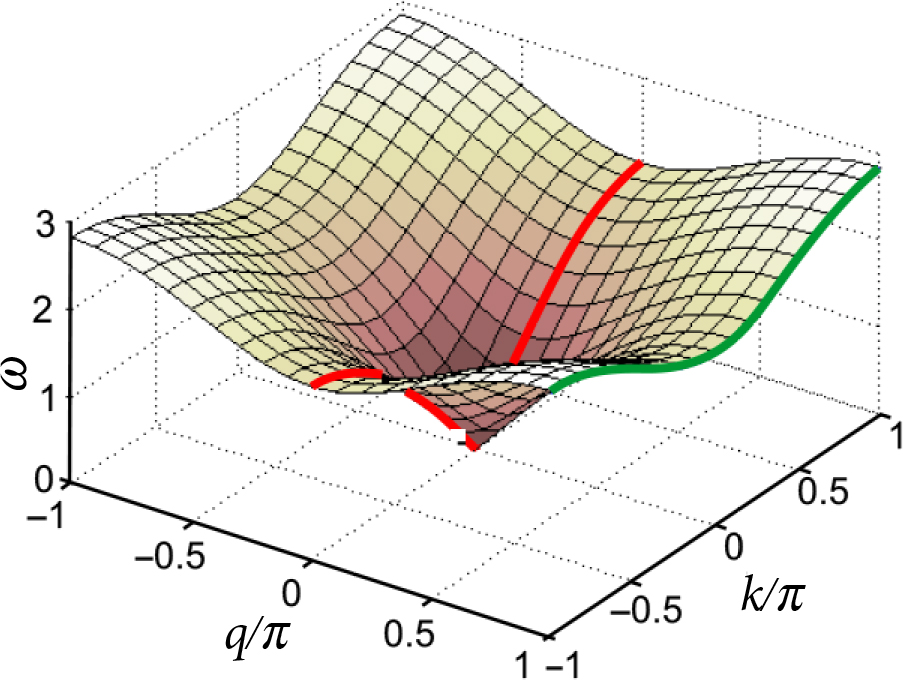Fig. 4. (Coloured online) Wave spectrum in the lattice without SLC. Mini-bands that appear in the case of N = 2 are indicated by red (middle) and green (side) solid curves.

However, as seen in Fig. 1, the vertical translation symmetry is partially preserved: the lattice can be translated vertically by N unit cells enabling us to use the analogue to the known Bloch’s theorem in solid state physics, and to assume the site potential

${U}_{n,m}={\text{e}}^{\text{i}qn}{V}_{n,m},\text{\hspace{0.17em}}\text{\hspace{0.17em}}\text{\hspace{0.17em}}\text{\hspace{0.17em}}\text{\hspace{0.17em}}\text{\hspace{0.17em}}\text{\hspace{0.17em}}\text{\hspace{0.17em}}\text{\hspace{0.17em}}\text{\hspace{0.17em}}\text{\hspace{0.17em}}\text{\hspace{0.17em}}\text{\hspace{0.17em}}\text{\hspace{0.17em}}\text{\hspace{0.17em}}\text{\hspace{0.17em}}\text{\hspace{0.17em}}\text{\hspace{0.17em}}\text{\hspace{0.17em}}\text{\hspace{0.17em}}\text{\hspace{0.17em}}\text{\hspace{0.17em}}\text{\hspace{0.17em}}\text{\hspace{0.17em}}\text{\hspace{0.17em}}\text{\hspace{0.17em}}\left(7\right)$

where the amplitude function satisfies the condition

${V}_{n+N,m}={V}_{n,m}\text{\hspace{0.17em}}\text{\hspace{0.17em}}\text{\hspace{0.17em}}\text{\hspace{0.17em}}\text{\hspace{0.17em}}\text{\hspace{0.17em}}\text{\hspace{0.17em}}\text{\hspace{0.17em}}\text{\hspace{0.17em}}\text{\hspace{0.17em}}\text{\hspace{0.17em}}\text{\hspace{0.17em}}\text{\hspace{0.17em}}\text{\hspace{0.17em}}\text{\hspace{0.17em}}\text{\hspace{0.17em}}\text{\hspace{0.17em}}\text{\hspace{0.17em}}\text{\hspace{0.17em}}\text{\hspace{0.17em}}\text{\hspace{0.17em}}\text{\hspace{0.17em}}\text{\hspace{0.17em}}\text{\hspace{0.17em}}\text{\hspace{0.17em}}\text{\hspace{0.17em}}\text{\hspace{0.17em}}\text{\hspace{0.17em}}\left(8\right)$

of periodicity in the vertical direction. That enables us to transform the wave scattering problem defined in the infinite plane to the problem of wave propagation in the N-wide horizontal strip (0 ≤ n < N) with a single SLC at the site n = = 0.

We restrict our consideration to the case with the incident wave coming from the left side of the strip with the q = 0 wave vector component, that enabling us to assume the periodicity of the site potential

${U}_{N,m}\text{\hspace{0.17em}}={U}_{0,m}.\text{\hspace{0.17em}}\text{\hspace{0.17em}}\text{\hspace{0.17em}}\text{\hspace{0.17em}}\text{\hspace{0.17em}}\text{\hspace{0.17em}}\text{\hspace{0.17em}}\text{\hspace{0.17em}}\text{\hspace{0.17em}}\text{\hspace{0.17em}}\text{\hspace{0.17em}}\text{\hspace{0.17em}}\text{\hspace{0.17em}}\text{\hspace{0.17em}}\text{\hspace{0.17em}}\text{\hspace{0.17em}}\text{\hspace{0.17em}}\text{\hspace{0.17em}}\text{\hspace{0.17em}}\text{\hspace{0.17em}}\text{\hspace{0.17em}}\text{\hspace{0.17em}}\text{\hspace{0.17em}}\text{\hspace{0.17em}}\text{\hspace{0.17em}}\text{\hspace{0.17em}}\text{\hspace{0.17em}}\text{\hspace{0.17em}}\left(9\right)$

It is evident from Fig. 1 that the lattice together with the horizontal incident homogeneous wave is invariant in respect of the vertical inversion (→ –n), and that enables us to restrict the consideration to even functions assuming that

${U}_{-n,m}\text{\hspace{0.17em}}={U}_{n,m}.\text{\hspace{0.17em}}\text{\hspace{0.17em}}\text{\hspace{0.17em}}\text{\hspace{0.17em}}\text{\hspace{0.17em}}\text{\hspace{0.17em}}\text{\hspace{0.17em}}\text{\hspace{0.17em}}\text{\hspace{0.17em}}\text{\hspace{0.17em}}\text{\hspace{0.17em}}\text{\hspace{0.17em}}\text{\hspace{0.17em}}\text{\hspace{0.17em}}\text{\hspace{0.17em}}\text{\hspace{0.17em}}\text{\hspace{0.17em}}\text{\hspace{0.17em}}\text{\hspace{0.17em}}\text{\hspace{0.17em}}\text{\hspace{0.17em}}\text{\hspace{0.17em}}\text{\hspace{0.17em}}\text{\hspace{0.17em}}\text{\hspace{0.17em}}\text{\hspace{0.17em}}\text{\hspace{0.17em}}\text{\hspace{0.17em}}\left(10\right)$

As for the symmetry in respect of the horizontal translation, it can be restored in a standard way, dividing the solution of the scattering problem into three regions: the left one where m ≤ –1, the right one where m ≥ 1, and the interface m = 0. In the left and right regions the potential satisfies the same Eq. (2) with W = 0, and consequently, we have the same dispersion relation (6). However, it will be slightly cut down due to the incident wave with q = 0 and conditions (9) and (10).

In order to take these restrictions automatically into account we replace the vertical exponent in Eq. (5) by cosine choosing the following function for the potential:

${U}_{n,m}={\text{e}}^{\text{i}km}\text{\hspace{0.17em}}\mathrm{cos}\left(qn\right).\text{\hspace{0.17em}}\text{\hspace{0.17em}}\text{\hspace{0.17em}}\text{\hspace{0.17em}}\text{\hspace{0.17em}}\text{\hspace{0.17em}}\text{\hspace{0.17em}}\text{\hspace{0.17em}}\text{\hspace{0.17em}}\text{\hspace{0.17em}}\text{\hspace{0.17em}}\text{\hspace{0.17em}}\text{\hspace{0.17em}}\text{\hspace{0.17em}}\text{\hspace{0.17em}}\text{\hspace{0.17em}}\text{\hspace{0.17em}}\text{\hspace{0.17em}}\left(11\right)$

It satisfies Eq. (2) in both left and right regions, and the condition (10). In order to satisfy the condition (9) of vertical periodicity

$\mathrm{cos}\left(0\right)=\mathrm{cos}\left(Nq\right)\text{\hspace{0.17em}}\text{\hspace{0.17em}}\text{\hspace{0.17em}}\text{\hspace{0.17em}}\text{\hspace{0.17em}}\text{\hspace{0.17em}}\text{\hspace{0.17em}}\text{\hspace{0.17em}}\text{\hspace{0.17em}}\text{\hspace{0.17em}}\text{\hspace{0.17em}}\text{\hspace{0.17em}}\text{\hspace{0.17em}}\text{\hspace{0.17em}}\text{\hspace{0.17em}}\text{\hspace{0.17em}}\text{\hspace{0.17em}}\text{\hspace{0.17em}}\text{\hspace{0.17em}}\text{\hspace{0.17em}}\text{\hspace{0.17em}}\left(12\right)$

we have to choose the following values of the vertical wave vector component:

${N}_{{q}_{j}}=\text{\hspace{0.17em}}2\pi j,\text{\hspace{0.17em}}\text{\hspace{0.17em}}\text{\hspace{0.17em}}\text{\hspace{0.17em}}\text{\hspace{0.17em}}\text{\hspace{0.17em}}\text{\hspace{0.17em}}{q}_{j}=\frac{2\pi }{N}\text{\hspace{0.17em}}j.\text{\hspace{0.17em}}\text{\hspace{0.17em}}\text{\hspace{0.17em}}\text{\hspace{0.17em}}\text{\hspace{0.17em}}\text{\hspace{0.17em}}\text{\hspace{0.17em}}\text{\hspace{0.17em}}\text{\hspace{0.17em}}\text{\hspace{0.17em}}\text{\hspace{0.17em}}\text{\hspace{0.17em}}\text{\hspace{0.17em}}\text{\hspace{0.17em}}\left(13\right)$

Here symbol j stands for a non-negative integer. Due to this q quantization the previous spectrum is split into mini-bands. For instance, in the case of N = 2 they are shown in Fig. 4 by two solid (red and green) curves.

## 5. Wave scattering

Now we shall formulate the scattering problem using the eigenfunctions introduced at the end of the previous section. It is rather convenient due to their orthogonality and because the number of them is about twice less than the number of sites across the strip. As we need only the even functions, this number reads

$M=\left\{{}_{\left(N+1\right)/2,\text{\hspace{0.17em}}\text{\hspace{0.17em}}\text{\hspace{0.17em}}\text{\hspace{0.17em}}\text{\hspace{0.17em}}\text{\hspace{0.17em}}\text{\hspace{0.17em}}\text{\hspace{0.17em}}N\text{\hspace{0.17em}}\text{odd}\text{.}}^{N/2+1,\text{\hspace{0.17em}}\text{\hspace{0.17em}}\text{\hspace{0.17em}}\text{\hspace{0.17em}}\text{\hspace{0.17em}}\text{\hspace{0.17em}}\text{\hspace{0.17em}}\text{\hspace{0.17em}}\text{\hspace{0.17em}}\text{\hspace{0.17em}}\text{\hspace{0.17em}}\text{\hspace{0.17em}}\text{\hspace{0.17em}}N\text{\hspace{0.17em}}\text{even,}}\text{\hspace{0.17em}}\text{\hspace{0.17em}}\text{\hspace{0.17em}}\text{\hspace{0.17em}}\text{\hspace{0.17em}}\text{\hspace{0.17em}}\text{\hspace{0.17em}}\text{\hspace{0.17em}}\text{\hspace{0.17em}}\text{\hspace{0.17em}}\text{\hspace{0.17em}}\text{\hspace{0.17em}}\text{\hspace{0.17em}}\text{\hspace{0.17em}}\text{\hspace{0.17em}}\text{\hspace{0.17em}}\text{\hspace{0.17em}}\text{\hspace{0.17em}}\text{\hspace{0.17em}}\text{\hspace{0.17em}}\text{\hspace{0.17em}}\text{\hspace{0.17em}}\text{\hspace{0.17em}}\left(14\right)$

According to the standard wave scattering theory we choose the potential in the left (l) m ≤ –1 and right (r) m ≥ 1 regions as follows:

${U}_{n,m}^{\left(l\right)}\text{\hspace{0.17em}}={\text{e}}^{\text{i}{k}_{0}m}{\psi }_{0}\left(n\right)+\sum _{j=0}^{M-1}{r}_{j}{\text{e}}^{-\text{i}{k}_{j}m}{\psi }_{j}\left(n\right),\text{\hspace{0.17em}}\text{\hspace{0.17em}}\text{\hspace{0.17em}}\text{\hspace{0.17em}}\text{\hspace{0.17em}}\text{\hspace{0.17em}}\text{\hspace{0.17em}}\text{\hspace{0.17em}}\text{\hspace{0.17em}}\text{\hspace{0.17em}}\text{\hspace{0.17em}}\text{\hspace{0.17em}}\text{\hspace{0.17em}}\text{\hspace{0.17em}}\text{\hspace{0.17em}}\text{\hspace{0.17em}}\text{\hspace{0.17em}}\text{\hspace{0.17em}}\text{\hspace{0.17em}}\text{\hspace{0.17em}}\text{\hspace{0.17em}}\text{\hspace{0.17em}}\left(15\text{a}\right)$
${U}_{n,m}^{\left(r\right)}\text{\hspace{0.17em}}=\sum _{j=0}^{M-1}{t}_{j}{\text{e}}^{-\text{i}{k}_{j}m}{\psi }_{j}\left(n\right).\text{\hspace{0.17em}}\text{\hspace{0.17em}}\text{\hspace{0.17em}}\text{\hspace{0.17em}}\text{\hspace{0.17em}}\text{\hspace{0.17em}}\text{\hspace{0.17em}}\text{\hspace{0.17em}}\text{\hspace{0.17em}}\text{\hspace{0.17em}}\text{\hspace{0.17em}}\text{\hspace{0.17em}}\text{\hspace{0.17em}}\text{\hspace{0.17em}}\text{\hspace{0.17em}}\text{\hspace{0.17em}}\text{\hspace{0.17em}}\text{\hspace{0.17em}}\text{\hspace{0.17em}}\text{\hspace{0.17em}}\text{\hspace{0.17em}}\text{\hspace{0.17em}}\text{\hspace{0.17em}}\text{\hspace{0.17em}}\text{\hspace{0.17em}}\text{\hspace{0.17em}}\text{\hspace{0.17em}}\text{\hspace{0.17em}}\text{\hspace{0.17em}}\text{\hspace{0.17em}}\text{\hspace{0.17em}}\text{\hspace{0.17em}}\text{\hspace{0.17em}}\text{\hspace{0.17em}}\text{\hspace{0.17em}}\text{\hspace{0.17em}}\text{\hspace{0.17em}}\text{\hspace{0.17em}}\text{\hspace{0.17em}}\left(15\text{b}\right)\text{\hspace{0.17em}}\text{\hspace{0.17em}}\text{\hspace{0.17em}}\text{\hspace{0.17em}}\text{\hspace{0.17em}}$

Here the wave vector component kj is defined by the dispersion relation (6)

$\mathrm{sin}\left({k}_{j}/2\right)=\sqrt{{\omega }^{2}/4-{\mathrm{sin}}^{2}\left({q}_{j}/2\right)},\text{\hspace{0.17em}}\text{\hspace{0.17em}}\text{\hspace{0.17em}}\text{\hspace{0.17em}}\text{\hspace{0.17em}}\text{\hspace{0.17em}}\text{\hspace{0.17em}}\text{\hspace{0.17em}}\text{\hspace{0.17em}}\text{\hspace{0.17em}}\text{\hspace{0.17em}}\text{\hspace{0.17em}}\text{\hspace{0.17em}}\text{\hspace{0.17em}}\text{\hspace{0.17em}}\text{\hspace{0.17em}}\text{\hspace{0.17em}}\text{\hspace{0.17em}}\text{\hspace{0.17em}}\text{\hspace{0.17em}}\text{\hspace{0.17em}}\text{\hspace{0.17em}}\text{\hspace{0.17em}}\text{\hspace{0.17em}}\text{\hspace{0.17em}}\text{\hspace{0.17em}}\left(16\right)$

and the symbol

${\psi }_{j}\left(n\right)={A}_{j}\mathrm{cos}\left({q}_{j}n\right)\text{\hspace{0.17em}}\text{\hspace{0.17em}}\text{\hspace{0.17em}}\text{\hspace{0.17em}}\text{\hspace{0.17em}}\text{\hspace{0.17em}}\text{\hspace{0.17em}}\text{\hspace{0.17em}}\text{\hspace{0.17em}}\text{\hspace{0.17em}}\text{\hspace{0.17em}}\text{\hspace{0.17em}}\text{\hspace{0.17em}}\text{\hspace{0.17em}}\text{\hspace{0.17em}}\text{\hspace{0.17em}}\text{\hspace{0.17em}}\text{\hspace{0.17em}}\text{\hspace{0.17em}}\text{\hspace{0.17em}}\text{\hspace{0.17em}}\text{\hspace{0.17em}}\text{\hspace{0.17em}}\text{\hspace{0.17em}}\text{\hspace{0.17em}}\text{\hspace{0.17em}}\text{\hspace{0.17em}}\text{\hspace{0.17em}}\text{\hspace{0.17em}}\text{\hspace{0.17em}}\text{\hspace{0.17em}}\text{\hspace{0.17em}}\text{\hspace{0.17em}}\text{\hspace{0.17em}}\text{\hspace{0.17em}}\text{\hspace{0.17em}}\left(17\right)$

stands for the normalized transverse eigenfunction. Its norm reads

${A}_{j}=\left\{{}_{{\left(N/2\right)}^{-1/2},\text{\hspace{0.17em}}\text{\hspace{0.17em}}\text{\hspace{0.17em}}\text{\hspace{0.17em}}\text{\hspace{0.17em}}\text{\hspace{0.17em}}\text{\hspace{0.17em}}\text{\hspace{0.17em}}\text{\hspace{0.17em}}\text{\hspace{0.17em}}\text{\hspace{0.17em}}\text{\hspace{0.17em}}\text{\hspace{0.17em}}\text{\hspace{0.17em}}\text{\hspace{0.17em}}\text{\hspace{0.17em}}{q}_{j}\ne 0,\pi .}^{{N}^{-1/2},\text{\hspace{0.17em}}\text{\hspace{0.17em}}\text{\hspace{0.17em}}\text{\hspace{0.17em}}\text{\hspace{0.17em}}\text{\hspace{0.17em}}\text{\hspace{0.17em}}\text{\hspace{0.17em}}\text{\hspace{0.17em}}\text{\hspace{0.17em}}\text{\hspace{0.17em}}\text{\hspace{0.17em}}\text{\hspace{0.17em}}\text{\hspace{0.17em}}\text{\hspace{0.17em}}\text{\hspace{0.17em}}\text{\hspace{0.17em}}\text{\hspace{0.17em}}\text{\hspace{0.17em}}\text{\hspace{0.17em}}\text{\hspace{0.17em}}\text{\hspace{0.17em}}\text{\hspace{0.17em}}\text{\hspace{0.17em}}\text{\hspace{0.17em}}\text{\hspace{0.17em}}\text{\hspace{0.17em}}\text{\hspace{0.17em}}{q}_{j}=0,\pi ,}\text{\hspace{0.17em}}\text{\hspace{0.17em}}\text{\hspace{0.17em}}\text{\hspace{0.17em}}\text{\hspace{0.17em}}\text{\hspace{0.17em}}\text{\hspace{0.17em}}\text{\hspace{0.17em}}\text{\hspace{0.17em}}\text{\hspace{0.17em}}\text{\hspace{0.17em}}\text{\hspace{0.17em}}\text{\hspace{0.17em}}\text{\hspace{0.17em}}\text{\hspace{0.17em}}\text{\hspace{0.17em}}\text{\hspace{0.17em}}\text{\hspace{0.17em}}\text{\hspace{0.17em}}\text{\hspace{0.17em}}\text{\hspace{0.17em}}\text{\hspace{0.17em}}\text{\hspace{0.17em}}\text{\hspace{0.17em}}\text{\hspace{0.17em}}\text{\hspace{0.17em}}\text{\hspace{0.17em}}\left(18\right)$

In the left region the potential is composed of the incident and reflected waves, while in the right region there are transmitted waves. The number of reflected waves coincides with the number of transmitted ones, and it is equal to the number of minibands in the spectrum of the strip.

These two functions have to be matched at the interface m = 0 where SLC is located. Rewriting Eq. (2) for the particular m = 1 and m = –1 cases it is easy to notice that they contain Un,0 value. Consequently, this value should be the same for both functions defined in the left and right regions,

${U}_{n,0}^{\left(l\right)}\text{\hspace{0.17em}}={U}_{n,0}^{\left(r\right)},\text{\hspace{0.17em}}\text{\hspace{0.17em}}\text{\hspace{0.17em}}\text{\hspace{0.17em}}\text{\hspace{0.17em}}\text{\hspace{0.17em}}\text{\hspace{0.17em}}\text{\hspace{0.17em}}\text{\hspace{0.17em}}\text{\hspace{0.17em}}\text{\hspace{0.17em}}\text{\hspace{0.17em}}\text{\hspace{0.17em}}\text{\hspace{0.17em}}\text{\hspace{0.17em}}\text{\hspace{0.17em}}\text{\hspace{0.17em}}\text{\hspace{0.17em}}\text{\hspace{0.17em}}\text{\hspace{0.17em}}\text{\hspace{0.17em}}\text{\hspace{0.17em}}\text{\hspace{0.17em}}\text{\hspace{0.17em}}\text{\hspace{0.17em}}\text{\hspace{0.17em}}\text{\hspace{0.17em}}\text{\hspace{0.17em}}\text{\hspace{0.17em}}\text{\hspace{0.17em}}\text{\hspace{0.17em}}\text{\hspace{0.17em}}\text{\hspace{0.17em}}\text{\hspace{0.17em}}\text{\hspace{0.17em}}\left(19\right)$

giving us the first boundary condition. Inserting into it expressions (15) we obtain the following set of equations:

${\psi }_{0}\left(n\right)+\sum _{j=0}^{M-1}{r}_{j}{\psi }_{j}\left(n\right)=\sum _{j=0}^{M-1}{t}_{j}{\psi }_{j}\left(n\right),\text{\hspace{0.17em}}\text{\hspace{0.17em}}\text{\hspace{0.17em}}\text{\hspace{0.17em}}\text{\hspace{0.17em}}\text{\hspace{0.17em}}\text{\hspace{0.17em}}\text{\hspace{0.17em}}\text{\hspace{0.17em}}\text{\hspace{0.17em}}\text{\hspace{0.17em}}\text{\hspace{0.17em}}\text{\hspace{0.17em}}\text{\hspace{0.17em}}\text{\hspace{0.17em}}\text{\hspace{0.17em}}\text{\hspace{0.17em}}\left(20\right)$

which due to the orthogonality of functions ψj (17) immediately converts itself into the following simple relations between the amplitudes of transmitted and reflected waves:

${r}_{0}={t}_{0}-1,\text{\hspace{0.17em}}\text{\hspace{0.17em}}\text{\hspace{0.17em}}\text{\hspace{0.17em}}\text{\hspace{0.17em}}\text{\hspace{0.17em}}\text{\hspace{0.17em}}\text{\hspace{0.17em}}\text{\hspace{0.17em}}\text{\hspace{0.17em}}\text{\hspace{0.17em}}\text{\hspace{0.17em}}\text{\hspace{0.17em}}\text{\hspace{0.17em}}\text{\hspace{0.17em}}\text{\hspace{0.17em}}\text{\hspace{0.17em}}\text{\hspace{0.17em}}\text{\hspace{0.17em}}\text{\hspace{0.17em}}\text{\hspace{0.17em}}\text{\hspace{0.17em}}\text{\hspace{0.17em}}\text{\hspace{0.17em}}\text{\hspace{0.17em}}\text{\hspace{0.17em}}\text{\hspace{0.17em}}\text{\hspace{0.17em}}\left(21\text{a}\right)$
${r}_{j}={t}_{j},\text{\hspace{0.17em}}\text{\hspace{0.17em}}\text{\hspace{0.17em}}\text{\hspace{0.17em}}\text{\hspace{0.17em}}\text{\hspace{0.17em}}\text{\hspace{0.17em}}\text{\hspace{0.17em}}\text{\hspace{0.17em}}\text{\hspace{0.17em}}j\ne 0,\text{\hspace{0.17em}}\text{\hspace{0.17em}}\text{\hspace{0.17em}}\text{\hspace{0.17em}}\text{\hspace{0.17em}}\text{\hspace{0.17em}}\text{\hspace{0.17em}}\text{\hspace{0.17em}}\text{\hspace{0.17em}}\text{\hspace{0.17em}}\text{\hspace{0.17em}}\text{\hspace{0.17em}}\text{\hspace{0.17em}}\text{\hspace{0.17em}}\text{\hspace{0.17em}}\left(21\text{b}\right)$

and in this way the number of amplitudes that we are looking for is reduced twice.

In order to define the remaining part of the amplitudes we need some additional boundary conditions. We obtain them just rewriting Eq. (2) for m = 0:

$\begin{array}{l}\left({\omega }^{2}-4\right)\text{\hspace{0.17em}}{U}_{n,0}^{\left(r\right)}+{U}_{n,-1}^{\left(l\right)}+{U}_{n,1}^{\left(r\right)}+{U}_{n,-1,0}^{\left(r\right)}\text{\hspace{0.17em}}+{U}_{n,+1,0}^{\left(r\right)}\\ ={\delta }_{n,0}W{U}_{0,0}^{\left(r\right)},\text{\hspace{0.17em}}\text{\hspace{0.17em}}\text{\hspace{0.17em}}\text{\hspace{0.17em}}\text{\hspace{0.17em}}\text{\hspace{0.17em}}\text{\hspace{0.17em}}\text{\hspace{0.17em}}\text{\hspace{0.17em}}\text{\hspace{0.17em}}\text{\hspace{0.17em}}\text{\hspace{0.17em}}\text{\hspace{0.17em}}\text{\hspace{0.17em}}\text{\hspace{0.17em}}\text{\hspace{0.17em}}\text{\hspace{0.17em}}\text{\hspace{0.17em}}\text{\hspace{0.17em}}\text{\hspace{0.17em}}\text{\hspace{0.17em}}\text{\hspace{0.17em}}\text{\hspace{0.17em}}\text{\hspace{0.17em}}\text{\hspace{0.17em}}\text{\hspace{0.17em}}\text{\hspace{0.17em}}\text{\hspace{0.17em}}\text{\hspace{0.17em}}\text{\hspace{0.17em}}\text{\hspace{0.17em}}\text{\hspace{0.17em}}\text{\hspace{0.17em}}\text{\hspace{0.17em}}\text{\hspace{0.17em}}\text{\hspace{0.17em}}\text{\hspace{0.17em}}\text{\hspace{0.17em}}\text{\hspace{0.17em}}\text{\hspace{0.17em}}\text{\hspace{0.17em}}\text{\hspace{0.17em}}\text{\hspace{0.17em}}\text{\hspace{0.17em}}\text{\hspace{0.17em}}\text{\hspace{0.17em}}\text{\hspace{0.17em}}\text{\hspace{0.17em}}\text{\hspace{0.17em}}\text{\hspace{0.17em}}\text{\hspace{0.17em}}\text{\hspace{0.17em}}\text{\hspace{0.17em}}\text{\hspace{0.17em}}\text{\hspace{0.17em}}\text{\hspace{0.17em}}\text{\hspace{0.17em}}\text{\hspace{0.17em}}\text{\hspace{0.17em}}\text{\hspace{0.17em}}\text{\hspace{0.17em}}\text{\hspace{0.17em}}\text{\hspace{0.17em}}\text{\hspace{0.17em}}\text{\hspace{0.17em}}\text{\hspace{0.17em}}\text{\hspace{0.17em}}\text{\hspace{0.17em}}\text{\hspace{0.17em}}\text{\hspace{0.17em}}\text{\hspace{0.17em}}\text{\hspace{0.17em}}\text{\hspace{0.17em}}\text{\hspace{0.17em}}\text{\hspace{0.17em}}\text{\hspace{0.17em}}\text{\hspace{0.17em}}\text{\hspace{0.17em}}\text{\hspace{0.17em}}\text{\hspace{0.17em}}\text{\hspace{0.17em}}\text{\hspace{0.17em}}\text{\hspace{0.17em}}\text{\hspace{0.17em}}\text{\hspace{0.17em}}\text{\hspace{0.17em}}\text{\hspace{0.17em}}\text{\hspace{0.17em}}\text{\hspace{0.17em}}\text{\hspace{0.17em}}\text{\hspace{0.17em}}\text{\hspace{0.17em}}\text{\hspace{0.17em}}\text{\hspace{0.17em}}\text{\hspace{0.17em}}\text{\hspace{0.17em}}\left(22\right)\end{array}$

properly indicating the potential functions belonging to the left and right regions. This boundary condition can be transformed into a simpler form rewriting it as

$\begin{array}{l}\left({\omega }^{2}-4\right)\text{\hspace{0.17em}}{U}_{n,0}^{\left(r\right)}+{U}_{n,-1}^{\left(r\right)}+{U}_{n,1}^{\left(r\right)}+{U}_{n,-1,0}^{\left(r\right)}\text{\hspace{0.17em}}+{U}_{n,+1,0}^{\left(r\right)}\\ ={U}_{n,-1}^{\left(r\right)}-{U}_{n,-1}^{\left(l\right)}+{\delta }_{n,0}W{U}_{0,0}^{\left(r\right)},\text{\hspace{0.17em}}\text{\hspace{0.17em}}\text{\hspace{0.17em}}\text{\hspace{0.17em}}\text{\hspace{0.17em}}\text{\hspace{0.17em}}\text{\hspace{0.17em}}\text{\hspace{0.17em}}\text{\hspace{0.17em}}\text{\hspace{0.17em}}\text{\hspace{0.17em}}\text{\hspace{0.17em}}\text{\hspace{0.17em}}\text{\hspace{0.17em}}\text{\hspace{0.17em}}\text{\hspace{0.17em}}\text{\hspace{0.17em}}\text{\hspace{0.17em}}\text{\hspace{0.17em}}\text{\hspace{0.17em}}\text{\hspace{0.17em}}\text{\hspace{0.17em}}\text{\hspace{0.17em}}\text{\hspace{0.17em}}\text{\hspace{0.17em}}\text{\hspace{0.17em}}\text{\hspace{0.17em}}\text{\hspace{0.17em}}\text{\hspace{0.17em}}\text{\hspace{0.17em}}\text{\hspace{0.17em}}\text{\hspace{0.17em}}\text{\hspace{0.17em}}\text{\hspace{0.17em}}\text{\hspace{0.17em}}\text{\hspace{0.17em}}\text{\hspace{0.17em}}\text{\hspace{0.17em}}\text{\hspace{0.17em}}\text{\hspace{0.17em}}\text{\hspace{0.17em}}\text{\hspace{0.17em}}\text{\hspace{0.17em}}\text{\hspace{0.17em}}\text{\hspace{0.17em}}\text{\hspace{0.17em}}\text{\hspace{0.17em}}\text{\hspace{0.17em}}\text{\hspace{0.17em}}\text{\hspace{0.17em}}\text{\hspace{0.17em}}\text{\hspace{0.17em}}\text{\hspace{0.17em}}\text{\hspace{0.17em}}\text{\hspace{0.17em}}\text{\hspace{0.17em}}\text{\hspace{0.17em}}\text{\hspace{0.17em}}\text{\hspace{0.17em}}\left(23\right)\end{array}$

and paying attention that its left-hand side includes only the potential functions marked by (r) symbols, and, consequently, is zeroed due to Eq. (2). Thus, (15) functions have to satisfy the second boundary condition:

${U}_{n,-1}^{\left(l\right)}-{U}_{n,-1}^{\left(r\right)}={\delta }_{n,0}W{U}_{0,0}^{\left(r\right)},\text{\hspace{0.17em}}\text{\hspace{0.17em}}\text{\hspace{0.17em}}\text{\hspace{0.17em}}\text{\hspace{0.17em}}\text{\hspace{0.17em}}\text{\hspace{0.17em}}\text{\hspace{0.17em}}\text{\hspace{0.17em}}\text{\hspace{0.17em}}\text{\hspace{0.17em}}\text{\hspace{0.17em}}\text{\hspace{0.17em}}\text{\hspace{0.17em}}\text{\hspace{0.17em}}\text{\hspace{0.17em}}\text{\hspace{0.17em}}\text{\hspace{0.17em}}\text{\hspace{0.17em}}\text{\hspace{0.17em}}\text{\hspace{0.17em}}\text{\hspace{0.17em}}\text{\hspace{0.17em}}\text{\hspace{0.17em}}\text{\hspace{0.17em}}\text{\hspace{0.17em}}\left(24\right)$
${\text{e}}^{-\text{i}{k}_{0}}{\psi }_{0}\left(n\right)+\sum _{l=0}^{M-1}\left({r}_{l}{\text{e}}^{-\text{i}{k}_{l}}-{t}_{l}{\text{e}}^{-\text{i}{k}_{l}}\right){\psi }_{l}\left(n\right)={\delta }_{n,0}W\sum _{l=0}^{M-1}{t}_{l}{\psi }_{l}\left(0\right).\text{\hspace{0.17em}}\text{\hspace{0.17em}}\text{\hspace{0.17em}}\text{\hspace{0.17em}}\text{\hspace{0.17em}}\text{\hspace{0.17em}}\text{\hspace{0.17em}}\text{\hspace{0.17em}}\text{\hspace{0.17em}}\text{\hspace{0.17em}}\text{\hspace{0.17em}}\text{\hspace{0.17em}}\text{\hspace{0.17em}}\text{\hspace{0.17em}}\text{\hspace{0.17em}}\text{\hspace{0.17em}}\text{\hspace{0.17em}}\text{\hspace{0.17em}}\text{\hspace{0.17em}}\text{\hspace{0.17em}}\text{\hspace{0.17em}}\text{\hspace{0.17em}}\text{\hspace{0.17em}}\text{\hspace{0.17em}}\text{\hspace{0.17em}}\text{\hspace{0.17em}}\text{\hspace{0.17em}}\text{\hspace{0.17em}}\text{\hspace{0.17em}}\text{\hspace{0.17em}}\left(25\right)$

Now multiplying this equation by ψj(n), summing the result by n, using the orthogonality of eigenfunctions and Eq. (17) we obtain the equation

${\text{e}}^{-\text{i}{k}_{0}}{\delta }_{j,0}+\left({r}_{j}{\text{e}}^{-\text{i}{k}_{j}}-{t}_{l}{\text{e}}^{-\text{i}{k}_{j}}\right)=W{A}_{j}\sum _{l=0}^{M-1}{t}_{l}{A}_{l},\text{\hspace{0.17em}}\text{\hspace{0.17em}}\text{\hspace{0.17em}}\text{\hspace{0.17em}}\text{\hspace{0.17em}}\text{\hspace{0.17em}}\text{\hspace{0.17em}}\text{\hspace{0.17em}}\text{\hspace{0.17em}}\text{\hspace{0.17em}}\text{\hspace{0.17em}}\text{\hspace{0.17em}}\text{\hspace{0.17em}}\text{\hspace{0.17em}}\text{\hspace{0.17em}}\text{\hspace{0.17em}}\text{\hspace{0.17em}}\text{\hspace{0.17em}}\text{\hspace{0.17em}}\text{\hspace{0.17em}}\text{\hspace{0.17em}}\text{\hspace{0.17em}}\text{\hspace{0.17em}}\left(26\right)$

that together with Eqs. (21) gives us the final equation

$\left({t}_{j}-{\delta }_{j,0}\right)\mathrm{sin}\text{​}\text{\hspace{0.17em}}{k}_{j}\text{\hspace{0.17em}}=-\frac{\text{i}}{2}W{A}_{j}\sum _{l=0}^{M-1}{A}_{l}{t}_{l}\text{\hspace{0.17em}}\text{\hspace{0.17em}}\text{\hspace{0.17em}}\text{\hspace{0.17em}}\text{\hspace{0.17em}}\text{\hspace{0.17em}}\text{\hspace{0.17em}}\text{\hspace{0.17em}}\text{\hspace{0.17em}}\text{\hspace{0.17em}}\text{\hspace{0.17em}}\text{\hspace{0.17em}}\text{\hspace{0.17em}}\text{\hspace{0.17em}}\text{\hspace{0.17em}}\text{\hspace{0.17em}}\text{\hspace{0.17em}}\text{\hspace{0.17em}}\text{\hspace{0.17em}}\text{\hspace{0.17em}}\text{\hspace{0.17em}}\text{\hspace{0.17em}}\text{\hspace{0.17em}}\text{\hspace{0.17em}}\text{\hspace{0.17em}}\text{\hspace{0.17em}}\text{\hspace{0.17em}}\text{\hspace{0.17em}}\text{\hspace{0.17em}}\text{\hspace{0.17em}}\text{\hspace{0.17em}}\text{\hspace{0.17em}}\text{\hspace{0.17em}}\text{\hspace{0.17em}}\text{\hspace{0.17em}}\left(27\right)$

for the amplitudes of transmitted waves.

The obtained equations can be easily solved due to the same expression

$\eta =\sum _{l=0}^{M-1}{A}_{l}{t}_{l},\text{\hspace{0.17em}}\text{\hspace{0.17em}}\text{\hspace{0.17em}}\text{\hspace{0.17em}}\text{\hspace{0.17em}}\text{\hspace{0.17em}}\text{\hspace{0.17em}}\text{\hspace{0.17em}}\text{\hspace{0.17em}}\text{\hspace{0.17em}}\text{\hspace{0.17em}}\text{\hspace{0.17em}}\text{\hspace{0.17em}}\text{\hspace{0.17em}}\text{\hspace{0.17em}}\text{\hspace{0.17em}}\text{\hspace{0.17em}}\text{\hspace{0.17em}}\text{\hspace{0.17em}}\text{\hspace{0.17em}}\text{\hspace{0.17em}}\text{\hspace{0.17em}}\text{\hspace{0.17em}}\text{\hspace{0.17em}}\text{\hspace{0.17em}}\text{\hspace{0.17em}}\left(28\right)$

in the right-hand side of any of them. Indeed, expressing the transmission amplitudes in terms of this parameter

${t}_{j}={\delta }_{j,0}-\frac{\text{i}W{A}_{j}\eta }{2\mathrm{sin}{k}_{j}},\text{\hspace{0.17em}}\text{\hspace{0.17em}}\text{\hspace{0.17em}}\text{\hspace{0.17em}}\text{\hspace{0.17em}}\text{\hspace{0.17em}}\text{\hspace{0.17em}}\text{\hspace{0.17em}}\text{\hspace{0.17em}}\text{\hspace{0.17em}}\text{\hspace{0.17em}}\text{\hspace{0.17em}}\text{\hspace{0.17em}}\text{\hspace{0.17em}}\text{\hspace{0.17em}}\text{\hspace{0.17em}}\text{\hspace{0.17em}}\text{\hspace{0.17em}}\text{\hspace{0.17em}}\text{\hspace{0.17em}}\text{\hspace{0.17em}}\text{\hspace{0.17em}}\left(29\right)$

and inserting it back into Eq. (28), we obtain the equation

$\eta ={A}_{0}\frac{\text{i}W\eta }{2}\sum _{l=0}^{M-1}\frac{{A}_{l}^{2}}{\mathrm{sin}{k}_{l}}\text{\hspace{0.17em}}\text{\hspace{0.17em}}\text{\hspace{0.17em}}\text{\hspace{0.17em}}\text{\hspace{0.17em}}\text{\hspace{0.17em}}\text{\hspace{0.17em}}\text{\hspace{0.17em}}\text{\hspace{0.17em}}\text{\hspace{0.17em}}\text{\hspace{0.17em}}\text{\hspace{0.17em}}\text{\hspace{0.17em}}\text{\hspace{0.17em}}\text{\hspace{0.17em}}\text{\hspace{0.17em}}\text{\hspace{0.17em}}\text{\hspace{0.17em}}\text{\hspace{0.17em}}\text{\hspace{0.17em}}\text{\hspace{0.17em}}\text{\hspace{0.17em}}\left(30\right)$

for the parameter η. Solving Eq. (30) we arrive at the expression

$\eta ={A}_{0}{\left(1+\frac{\text{i}W}{2}\sum _{l=0}^{M-1}\frac{{A}_{l}^{2}}{\mathrm{sin}{k}_{l}}\right)}^{-1}\text{\hspace{0.17em}}\text{\hspace{0.17em}}\text{\hspace{0.17em}}\text{\hspace{0.17em}}\text{\hspace{0.17em}}\text{\hspace{0.17em}}\text{\hspace{0.17em}}\text{\hspace{0.17em}}\text{\hspace{0.17em}}\text{\hspace{0.17em}}\text{\hspace{0.17em}}\text{\hspace{0.17em}}\text{\hspace{0.17em}}\text{\hspace{0.17em}}\text{\hspace{0.17em}}\text{\hspace{0.17em}}\text{\hspace{0.17em}}\text{\hspace{0.17em}}\text{\hspace{0.17em}}\left(31\right)$

that together with Eq. (29) solves the scattering problem.

In the next section we present an illustration of the application of Eqs. (29) and (31) in the case when the waves are scattered by the simplest SLC composed of inductance L0 and capacitance C0 connected in series with the admittance

${Y}_{0}=\frac{i\omega }{{L}_{0}\left[{\omega }^{2}-1/\left({L}_{0}{C}_{0}\right)\right]}\text{\hspace{0.17em}}\text{\hspace{0.17em}}\text{\hspace{0.17em}}\text{\hspace{0.17em}}\text{\hspace{0.17em}}\text{\hspace{0.17em}}\text{\hspace{0.17em}}\text{\hspace{0.17em}}\text{\hspace{0.17em}}\text{\hspace{0.17em}}\text{\hspace{0.17em}}\text{\hspace{0.17em}}\text{\hspace{0.17em}}\text{\hspace{0.17em}}\text{\hspace{0.17em}}\text{\hspace{0.17em}}\text{\hspace{0.17em}}\text{\hspace{0.17em}}\text{\hspace{0.17em}}\text{\hspace{0.17em}}\text{\hspace{0.17em}}\text{\hspace{0.17em}}\text{\hspace{0.17em}}\text{\hspace{0.17em}}\left(32\right)$

and

$\begin{array}{l}W=\frac{{\omega }^{2}\left({\omega }^{2}-{\omega }_{0}^{2}+b\right)}{{\omega }^{2}-{\omega }_{0}^{2}},\\ {\omega }_{0}^{2}=1/\left({L}_{0}{C}_{0}\right),b=1/{L}_{0}.\text{\hspace{0.17em}}\text{\hspace{0.17em}}\text{\hspace{0.17em}}\text{\hspace{0.17em}}\text{\hspace{0.17em}}\text{\hspace{0.17em}}\text{\hspace{0.17em}}\text{\hspace{0.17em}}\text{\hspace{0.17em}}\text{\hspace{0.17em}}\text{\hspace{0.17em}}\text{\hspace{0.17em}}\text{\hspace{0.17em}}\text{\hspace{0.17em}}\text{\hspace{0.17em}}\text{\hspace{0.17em}}\text{\hspace{0.17em}}\text{\hspace{0.17em}}\text{\hspace{0.17em}}\text{\hspace{0.17em}}\text{\hspace{0.17em}}\text{\hspace{0.17em}}\text{\hspace{0.17em}}\text{\hspace{0.17em}}\text{\hspace{0.17em}}\text{\hspace{0.17em}}\text{\hspace{0.17em}}\text{\hspace{0.17em}}\text{\hspace{0.17em}}\left(33\right)\end{array}$

## 6. Results

In the most simple N = 1 case the result (squared modules of the transmitted and reflected wave amplitudes) is shown in Fig. 5. They correspond to the density of energy in waves. In this case they actually show the transmittance and reflectance

${T}_{0}={|{t}_{0}|}^{2},\text{\hspace{0.17em}}\text{\hspace{0.17em}}\text{\hspace{0.17em}}\text{\hspace{0.17em}}\text{\hspace{0.17em}}\text{\hspace{0.17em}}\text{\hspace{0.17em}}\text{\hspace{0.17em}}\text{\hspace{0.17em}}\text{\hspace{0.17em}}\text{\hspace{0.17em}}\text{\hspace{0.17em}}\text{\hspace{0.17em}}\text{\hspace{0.17em}}\text{\hspace{0.17em}}\text{\hspace{0.17em}}\text{\hspace{0.17em}}\text{\hspace{0.17em}}{R}_{0}={|{r}_{0}|}^{2}\text{\hspace{0.17em}}\text{\hspace{0.17em}}\text{\hspace{0.17em}}\text{\hspace{0.17em}}\text{\hspace{0.17em}}\text{\hspace{0.17em}}\text{\hspace{0.17em}}\text{\hspace{0.17em}}\text{\hspace{0.17em}}\text{\hspace{0.17em}}\text{\hspace{0.17em}}\text{\hspace{0.17em}}\text{\hspace{0.17em}}\text{\hspace{0.17em}}\text{\hspace{0.17em}}\text{\hspace{0.17em}}\text{\hspace{0.17em}}\text{\hspace{0.17em}}\text{\hspace{0.17em}}\left(34\right)$

because the incident, transmitted and reflected waves are of the same dominant mode, and consequently, they have the same group velocity ${v}_{0}^{\left(\text{gr}\right)}$ which reads

${{v}_{j}^{\left(\text{gr}\right)}\text{\hspace{0.17em}}=\text{\hspace{0.17em}}\frac{\text{d}\omega }{\text{d}k}|}_{k={k}_{j}}\text{\hspace{0.17em}}=\frac{\mathrm{sin}{k}_{j}}{\omega }.\text{\hspace{0.17em}}\text{\hspace{0.17em}}\text{\hspace{0.17em}}\text{\hspace{0.17em}}\text{\hspace{0.17em}}\text{\hspace{0.17em}}\text{\hspace{0.17em}}\text{\hspace{0.17em}}\text{\hspace{0.17em}}\text{\hspace{0.17em}}\text{\hspace{0.17em}}\text{\hspace{0.17em}}\text{\hspace{0.17em}}\text{\hspace{0.17em}}\text{\hspace{0.17em}}\text{\hspace{0.17em}}\text{\hspace{0.17em}}\text{\hspace{0.17em}}\text{\hspace{0.17em}}\text{\hspace{0.17em}}\text{\hspace{0.17em}}\text{\hspace{0.17em}}\text{\hspace{0.17em}}\text{\hspace{0.17em}}\text{\hspace{0.17em}}\text{\hspace{0.17em}}\text{\hspace{0.17em}}\left(35\right)$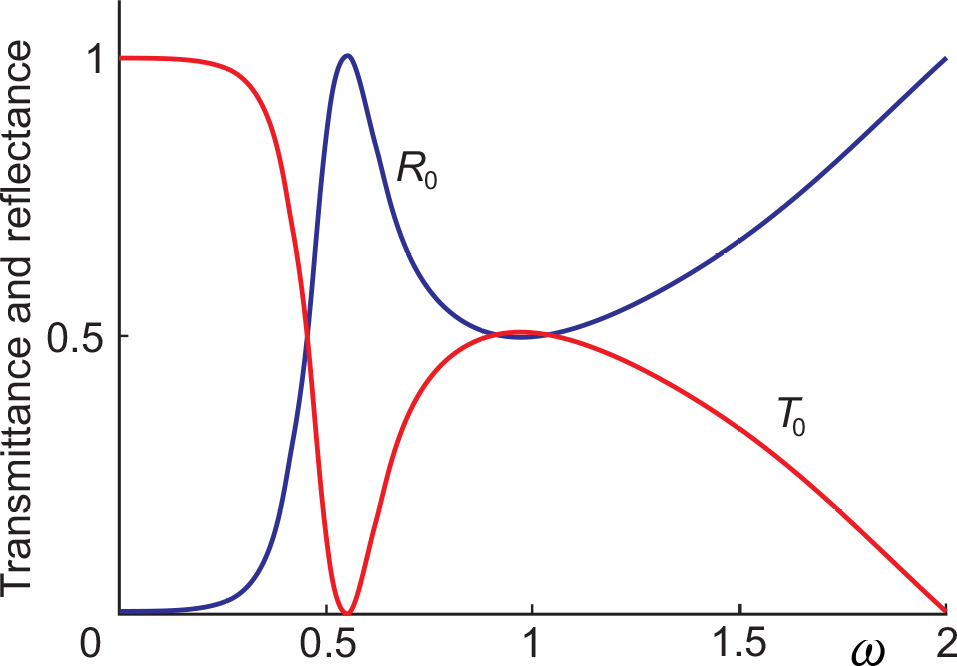Fig. 5. (Coloured online) Transmittance (a red curve) and reflectance (a blue curve) in the case N = 1: ω0 = 0.548, L0 = 2 (b = 0.5).

A single interesting point is a negative peak in transmission at about the resonance frequency of SLC ω0. It is quite expectable as in this case SLC is in every horizontal line of the lattice and, consequently, it is short-circuited at that resonant frequency. Note that the peak is negative and rather non-symmetric. In that sense it is an analogue to the well-known Fano resonance  that mainly manifests itself when there is some coupling between a discrete bound state (the resonance related to SLC in our case) and a continuum of states (the electric wave in the lattice itself). This negative peak is an analogue to the plasmonic mode in experiments with SRRs. If one likes to imitate more of these resonances, several SLC with different resonance frequencies can be connected in parallel.

In Fig. 6 the same transmittance (a red curve) and reflectance (a blue curve) of dominant modes are shown in the case of N = 2, namely, when the capacitances are replaced by SLCs in every second line. The plot is similar to that shown in Fig. 5. We see the same negative peak at almost the same position although slightly narrower. This behaviour is in agreement with the analytic estimations presented in Appendix A.

There is, however, a new point, namely, the excited mode amplitude t1, and, according to Eq. (21b), the reflected mode amplitude r1 coinciding with it. The matter is that in the case of N = 2 there are two minibands in the spectrum (see the inset in Fig. 6), and consequently, two transmitted and two reflected waves. The additional mode, however, is the evanescent wave because the excited mini-band does not overlap with the dominant one, and in the frequency range under the consideration it is characterised by the imaginary wave vector k1 component in Eq. (16). Being actually the interface waves they give no contribution to energy flow. Sometimes they are called the lattice waves due to their possible propagation only along the vertical SLC line. The closer the frequency to the bottom of the exited mini-band is, the larger the amplitude of the corresponding evanescent mode is. Note there is some resonance of the evanescent mode close to the negative peak of the transmitted dominant mode.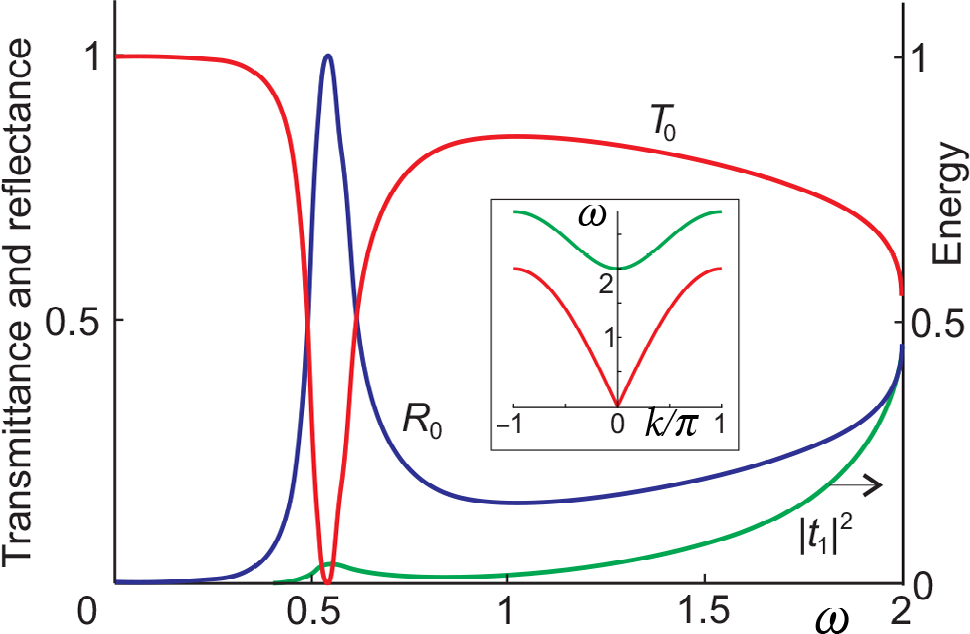Fig. 6. (Coloured online) The transmittance of the dominant mode T0 (a red curve), its reflectance R0 (a blue curve), and the energy density of the first excited mode |t1|2 (a green curve) in the case of N = 2. In the inset the spectrum consisting of two mini-bands is shown (see the spectrum in Fig. 4).

In the case of N = 3 the excited mini-band lowers itself (see the inset in Fig. 7) and overlaps with the dominant mini-band in the region above its cutoff frequency ω*. This fact changes the behaviour of the transmittance in the region ω ≥ ω*, as is seen in Fig. 7. Here the excited mode is propagating (contrary to the evanescent wave that is in the ω ≤ ω* region), and that is why it contributes to the transmittance which reads

${T}_{1}=\left({v}_{j}{}^{\left(\text{gr}\right)}/{v}_{0}{}^{\left(\text{gr}\right)}\right)\text{\hspace{0.17em}}{|{t}_{1}|}^{2},\text{\hspace{0.17em}}\text{\hspace{0.17em}}\text{\hspace{0.17em}}\text{\hspace{0.17em}}\text{\hspace{0.17em}}\text{\hspace{0.17em}}\text{\hspace{0.17em}}\text{\hspace{0.17em}}\text{\hspace{0.17em}}\text{\hspace{0.17em}}\text{\hspace{0.17em}}\text{\hspace{0.17em}}\text{\hspace{0.17em}}\text{\hspace{0.17em}}\text{\hspace{0.17em}}\text{\hspace{0.17em}}\text{\hspace{0.17em}}\text{\hspace{0.17em}}\text{\hspace{0.17em}}\text{\hspace{0.17em}}\text{\hspace{0.17em}}\text{\hspace{0.17em}}\text{\hspace{0.17em}}\text{\hspace{0.17em}}\text{\hspace{0.17em}}\text{\hspace{0.17em}}\text{\hspace{0.17em}}\text{\hspace{0.17em}}\text{\hspace{0.17em}}\text{\hspace{0.17em}}\text{\hspace{0.17em}}\text{\hspace{0.17em}}\text{\hspace{0.17em}}\left(36\right)$

and is shown in Fig. 7 by a green dotted curve. So, the total transmittance in the ω ≤ ω* region coincides with the transmittance of the dominant mode, while above the cutoff frequency ω* it consists of two parts T = T0 + T1 and is shown by a red dotted curve. The total reflectance can be obtained by means of vertical inversion of the total transmittance.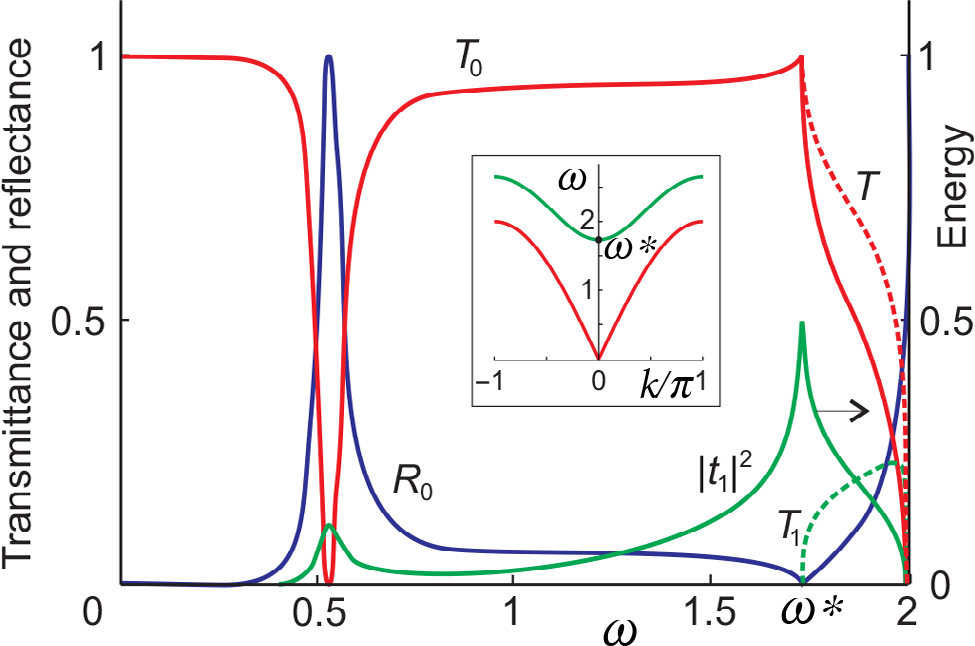Fig. 7. (Coloured online) The transmittance T0 (a red solid curve) and reflectance R0 (a blue solid curve) of the dominant mode, the energy density |t1|2 (a solid green curve) and transmittance T1 (a dotted green curve) of the first excited mode in the case of N = 3. The red dotted curve is the total transmittance T = T0 + T1. In the inset the spectrum consisting of two overlapping mini-bands is shown.

Note a few interesting points that are worth mentioning. One of them is the complete transmission (T = 1) at the cutoff frequency of the excited mode. It is an analogue to the complete tunnelling of a quantum mechanical particle through the discrete level. In the considered equivalent circuit the role of the discrete level is played by the compression of the density of states of actually a 1D wave motion in a strip at the cutoff frequency.

The second point is the essential asymmetry of the transmittance of the excited mode at its cutoff frequency where the evanescent lattice mode changes into the propagating wave. It properly correlates with the Wood’s result of anomaly dispersion .

The third point is a significant impact of the excited mode on the width of the negative transmission peak at the resonance SLC frequency. It is worth mentioning that such behaviour of plasmonic resonance modes was observed experimentally .

The main conclusions (the peculiarities in transmittance at cutoff frequency) are not restricted to the considered N = 3 case. That is illustrated in Fig. 8, where the densities of mode energies (see the inset) and the corresponding energy flows are shown in the case of N = 7.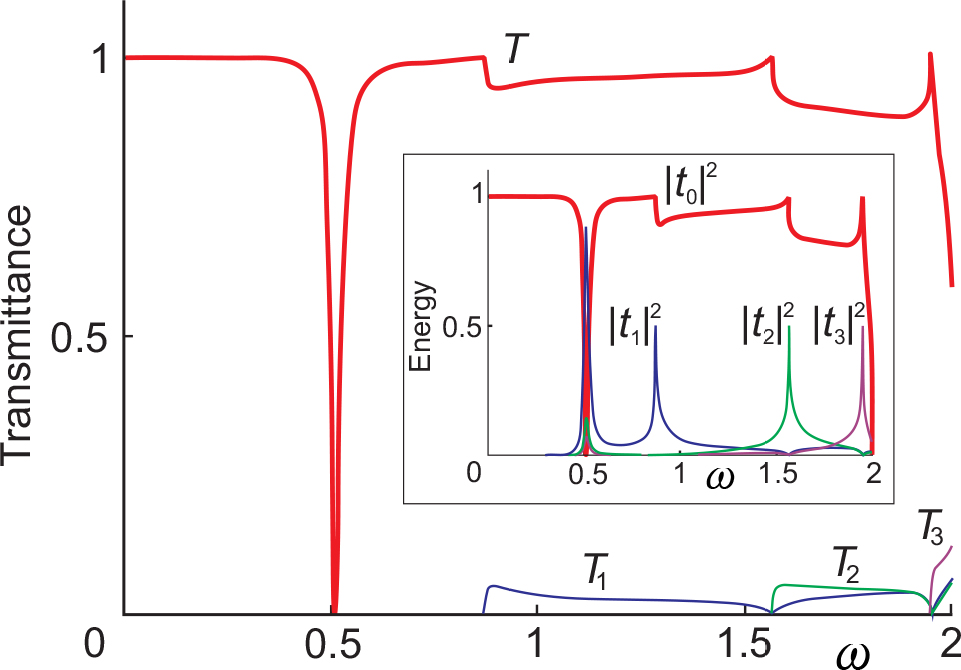Fig. 8. (Coloured online) Total transmittance T = T1 + T2 + T3 (a red curve), and the partial ones: T1 is a blue curve, T2 is a green curve, T3 is a violet curve in the case of N = 7. In the inset: energy densities of various modes.

As shown in Appendix A (see Eq. (A2)), in the limit of large N the characteristic frequencies of the complete wave transmission are the linear functions of parameter 1/N, which is an analogue to the inverse distance (1/L) between the neighbouring SLCs. This is illustrated by the contour plot of transmittance in Fig. 9 in the case of ω0 = 0.55, L0 = 3 and N changing from 2 to 501. Additional yellow solid lines in the figure correspond to the first three cutoff frequencies of mini-bands given by Eq. (A2).

Note that in the region of large N values these complete transmission frequencies (lattice modes) intersect the dashed horizontal line corresponding to the SLC resonance (an analogue to the plasmonic mode), demonstrating a typical anticrossing behaviour that was observed when investigating two-dimensional SRR arrays . One of these anticrossings is shown in detail in Fig. 10 where the transmittance plot in the ω–ω0 plane for the fixed N = 7 value is presented. It is clearly seen that the red solid line (representing the lattice mode) breaks off the continuous motion of the negative peak of SLC resonance, changing the roles of these two modes close to the intersection point. Besides, above the red line the peak of SLC resonance is wider and shallower.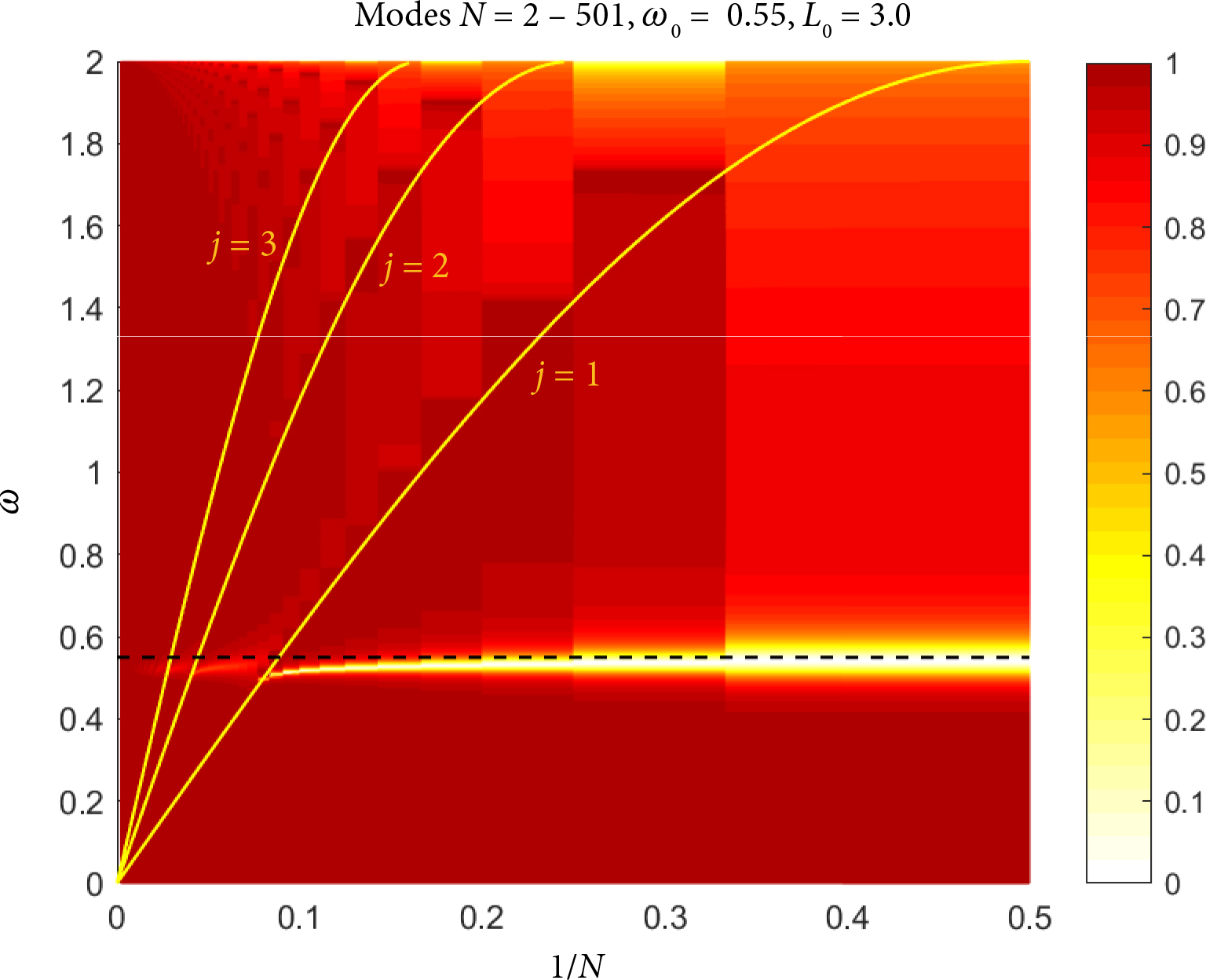Fig. 9. (Coloured online) Variation of transmittance in the ω–1/N plane. An additional black dashed line shows the resonance frequency of SLC and solid yellow lines correspond to the first three frequencies of complete transmission of the wave at the bottom of the excited mini-band.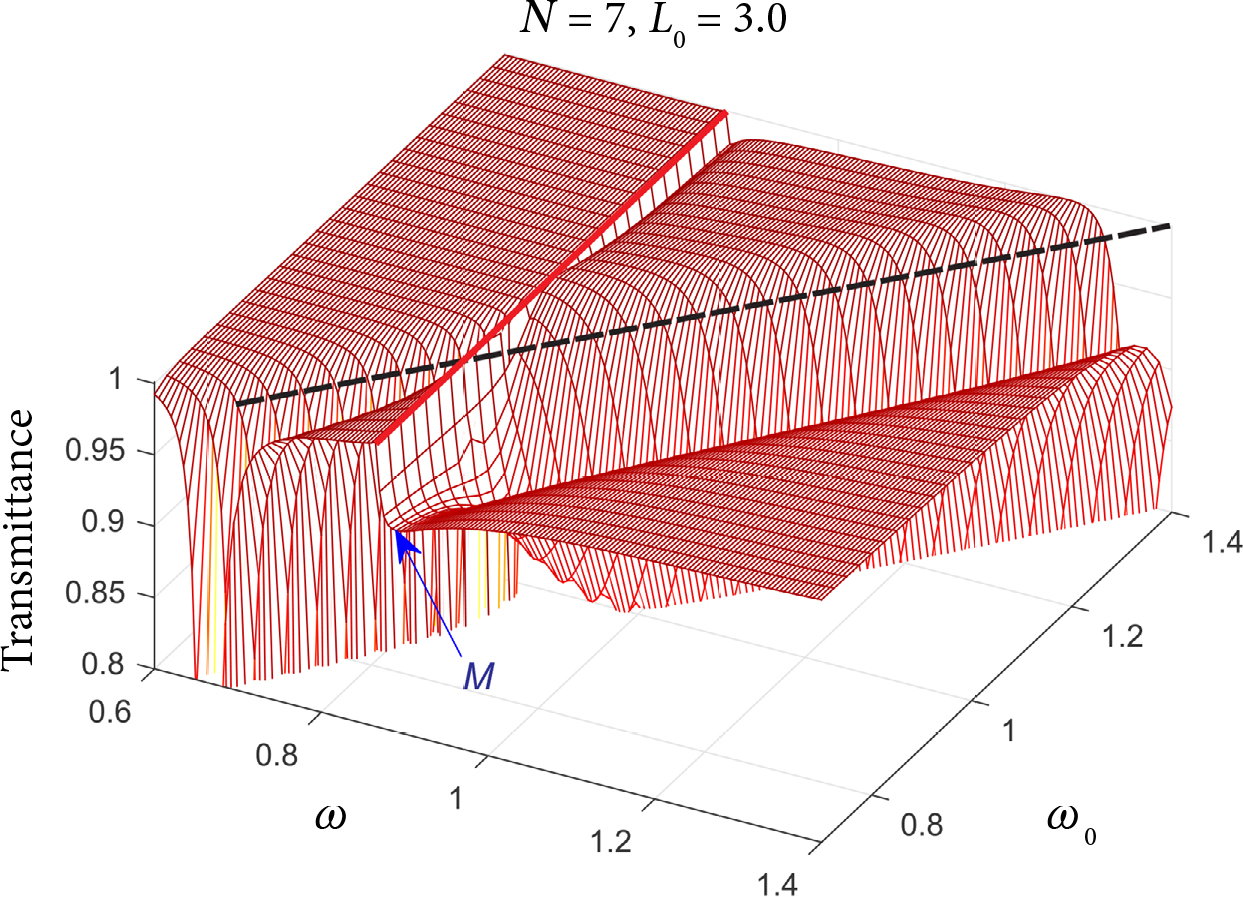Fig. 10. (Coloured online) Transmittance plot in the ω–ω0 plane showing the anti-crossing of the lattice mode (the complete transmission of the wave indicated by a red line) with SLC resonance (the total suppression of transmission indicated by a black dashed line) for N = 7 and L0 = 3. The letter M denotes the minimum adjacent to the transmission maximum.

## 7. Conclusions

We used a rather simple model, based on a circuit of periodic lumped elements, imitating an array of split-ring resonators. The periodicity of the series resonant LC circuit inserted into a chain of lumped elements allows us to transform a problem of diffraction in an infinite plane of lumped elements into a problem of wave transmission through the chain containing an LC circuit. The spectrum of the transmitted wave contains mini-bands, each of which generates one transmitted and reflected mode. The analytical solution of the problem clearly indicates two types of resonances. The reasons of their appearance are the same as in the experiments or modelling of arrays of split-ring resonators.

The first type of them is the resonance practically independent of 1/N and conditioned by the individual resonance of the inserted LC circuit. Its half-width depends on the distance between circuits, namely: the increase of distance leads to its decrease. Such a behaviour demonstrates that resonance of the LC circuit inserted in a lumped element chain differs from the standard energy absorption by the system with one degree of freedom. It is similar to the resonance scattering, the essence of which is constructive and destructive interference appearing due to interaction of propagating waves with the local oscillation of the LC circuit, having similarities with Fano resonance .

The position of the second type resonance linearly depends on 1/N for the large N. Its characteristic frequencies match to the bottom of each mini-band. Namely, at this frequency the energy density of the mini-band resonates demonstrating a symmetrical resonance peak. Contrary to it, the energy flow demonstrates a strongly antisymmetric behaviour, since the low frequency side of the peak corresponds to the local non-propagating evanescent mode, whereas the propagating mode corresponds to the high frequency side of the peak. This asymmetry is reflected on the fundamental mode and on the total transmittance as well. It is worthwhile to note that at the resonance frequency the full transmission of the fundamental mode is observed, the asymmetry of which can also be interpreted as Fano resonance. It appears due to wave interaction with the bottom of the mini-band where the peculiarity of the density of states is characteristic of the continuous spectrum.

At first sight the full transmission at the frequency of the second type resonance disagrees with experimental results [7, 13], where the decrease of the transmittance was observed depending on a distance between SRRs. On the one hand, in the considered model the distance between peaks is decreasing when N grows. Between the peaks the suppression of the transmission is observed that can be compared with the experimental data. On the other hand, one cannot expect to get a good quantitative coincidence since the model is too crude and Fano resonance is very sensitive to the tuning of the phase of interacting modes which in a two-dimensional model and a three-dimensional experiment can differ essentially.

## Acknowledgements

G. Š. has received funding from the European Social Fund (Project No. 09.3.3-LMT-K-712-02-0037) under Grant Agreement with the Research Council of Lithuania (LMTLT).

### Appendix A Analytical expressions and asymptotic estimations

The analytic solution Eqs. (29), (31) of the model wave scattering problem enables us to obtain some useful exact expressions and asymptotic evaluations. This section is devoted to derivation of them.

The easiest job is to establish the values of peaks. Indeed, let us assume that the frequency ωj corresponds to the bottom of the j mini-band. Then kj = 0 and sin kj = 0. Consequently, at this frequency the denominator in Eq. (31) turns into infinity, the parameter η is zeroed, and all amplitudes tj have to be zeroed as well. This consideration, however, holds only in the case of t0. According to Eq. (29) the second term of its right-hand side becomes equal to zero, and we have the unit value of the peak in the dominant transmission mode.

For the excited modes the abovementioned consideration is not correct. The matter is that in the case of tj (≠ 0) the infinity in the denominator of expression (31) is compensated by an equal to zero sin kj in the denominator of Eq. (29). That is why a more accurate calculation has to be carried out:

$\begin{array}{l}\underset{\omega \to {\omega }_{j}}{\mathrm{lim}}{t}_{j}=\underset{{k}_{j}\to 0}{\mathrm{lim}}\left[\frac{-\text{i}W{A}_{0}{A}_{j}}{2\mathrm{sin}{k}_{j}\left(1+iW{\sum }_{l=0}^{M-1}\frac{{A}_{l}^{2}}{2\mathrm{sin}{k}_{l}}\right)}\right]\\ \text{\hspace{0.17em}}\text{\hspace{0.17em}}\text{\hspace{0.17em}}\text{\hspace{0.17em}}\text{\hspace{0.17em}}\text{\hspace{0.17em}}\text{\hspace{0.17em}}\text{\hspace{0.17em}}\text{\hspace{0.17em}}\text{\hspace{0.17em}}\text{\hspace{0.17em}}\text{\hspace{0.17em}}\text{\hspace{0.17em}}\text{\hspace{0.17em}}\text{\hspace{0.17em}}\text{\hspace{0.17em}}\text{\hspace{0.17em}}\text{\hspace{0.17em}}\text{\hspace{0.17em}}\text{\hspace{0.17em}}\text{\hspace{0.17em}}\text{\hspace{0.17em}}\text{\hspace{0.17em}}\text{\hspace{0.17em}}\text{\hspace{0.17em}}\text{\hspace{0.17em}}\text{\hspace{0.17em}}\text{\hspace{0.17em}}\text{\hspace{0.17em}}\text{\hspace{0.17em}}\text{\hspace{0.17em}}\text{\hspace{0.17em}}\text{\hspace{0.17em}}\text{\hspace{0.17em}}\text{\hspace{0.17em}}\text{\hspace{0.17em}}\text{\hspace{0.17em}}\text{\hspace{0.17em}}\text{\hspace{0.17em}}\text{\hspace{0.17em}}\text{\hspace{0.17em}}\text{\hspace{0.17em}}\text{\hspace{0.17em}}\text{\hspace{0.17em}}\text{\hspace{0.17em}}\text{\hspace{0.17em}}\text{\hspace{0.17em}}\text{\hspace{0.17em}}\text{\hspace{0.17em}}\text{\hspace{0.17em}}\text{\hspace{0.17em}}\text{\hspace{0.17em}}\text{\hspace{0.17em}}\text{\hspace{0.17em}}\text{\hspace{0.17em}}\text{\hspace{0.17em}}\text{\hspace{0.17em}}\text{\hspace{0.17em}}\text{\hspace{0.17em}}\text{\hspace{0.17em}}\text{\hspace{0.17em}}\text{\hspace{0.17em}}\text{\hspace{0.17em}}\text{\hspace{0.17em}}\text{\hspace{0.17em}}\text{\hspace{0.17em}}\text{\hspace{0.17em}}\text{\hspace{0.17em}}\text{\hspace{0.17em}}\text{\hspace{0.17em}}\text{\hspace{0.17em}}\text{\hspace{0.17em}}\text{\hspace{0.17em}}\text{\hspace{0.17em}}\text{\hspace{0.17em}}\text{\hspace{0.17em}}\text{\hspace{0.17em}}\text{\hspace{0.17em}}\text{\hspace{0.17em}}\text{\hspace{0.17em}}\text{\hspace{0.17em}}\text{\hspace{0.17em}}\text{\hspace{0.17em}}\text{\hspace{0.17em}}\text{\hspace{0.17em}}\text{\hspace{0.17em}}\text{\hspace{0.17em}}\text{\hspace{0.17em}}\text{\hspace{0.17em}}\text{\hspace{0.17em}}\text{\hspace{0.17em}}\text{\hspace{0.17em}}\text{\hspace{0.17em}}\text{\hspace{0.17em}}\text{\hspace{0.17em}}\text{\hspace{0.17em}}\text{\hspace{0.17em}}\text{\hspace{0.17em}}\text{\hspace{0.17em}}\text{\hspace{0.17em}}\text{\hspace{0.17em}}\text{\hspace{0.17em}}\text{\hspace{0.17em}}\text{\hspace{0.17em}}\text{\hspace{0.17em}}\text{\hspace{0.17em}}\left(\text{A1}\right)\\ =\underset{{k}_{j}\to 0}{\mathrm{lim}}\left[\frac{-\text{i}W{A}_{0}{A}_{j}}{2\mathrm{sin}{k}_{j}+\text{i}W{N}_{j}^{2}}\right]=-\frac{{A}_{0}}{{A}_{j}}=-\frac{1}{\sqrt{2}},\end{array}$

and |tj|2 = 1/2, as seen in Figs. 6 and 7.

The peak frequencies of the full transmission immediately follow from the dispersion relation (6). As already mentioned, kj = 0 at the cutoff frequency of the excited mode. Then it follows from the dispersion relation that

${\omega }_{j}=2\mathrm{sin}\left(\frac{\pi }{N}j\right)\approx \frac{2\pi j}{N},\text{\hspace{0.17em}}\text{\hspace{0.17em}}\text{\hspace{0.17em}}\text{\hspace{0.17em}}\text{\hspace{0.17em}}\text{\hspace{0.17em}}\text{\hspace{0.17em}}\text{\hspace{0.17em}}\text{\hspace{0.17em}}\text{\hspace{0.17em}}\text{\hspace{0.17em}}\text{\hspace{0.17em}}\text{\hspace{0.17em}}\text{\hspace{0.17em}}\text{\hspace{0.17em}}\text{\hspace{0.17em}}\text{\hspace{0.17em}}\text{\hspace{0.17em}}\text{\hspace{0.17em}}\text{\hspace{0.17em}}\left(\text{A2}\right)$

which confirms that in the asymptotic region of large N every full transmission peak frequency ωj is a linear 1/N function.

The properties of the negative resonance peak at frequency ω0 are sensitive to a definite structure of SLC. In the considered case of admittance given by Eqs. (32) and (33) this resonance appears due to infinity in the denominator of Eq. (33). That is why it is convenient to present that equation in the form

$W=P/Q,\text{\hspace{0.17em}}\text{\hspace{0.17em}}\text{\hspace{0.17em}}\text{\hspace{0.17em}}\text{\hspace{0.17em}}\text{\hspace{0.17em}}\text{\hspace{0.17em}}\text{\hspace{0.17em}}\text{\hspace{0.17em}}\text{\hspace{0.17em}}\text{\hspace{0.17em}}\text{\hspace{0.17em}}\text{\hspace{0.17em}}\text{\hspace{0.17em}}\text{\hspace{0.17em}}\text{\hspace{0.17em}}\text{\hspace{0.17em}}\text{\hspace{0.17em}}\text{\hspace{0.17em}}\text{\hspace{0.17em}}\text{\hspace{0.17em}}\text{\hspace{0.17em}}\text{\hspace{0.17em}}\text{\hspace{0.17em}}\text{\hspace{0.17em}}\text{\hspace{0.17em}}\text{\hspace{0.17em}}\text{\hspace{0.17em}}\text{\hspace{0.17em}}\text{\hspace{0.17em}}\text{\hspace{0.17em}}\text{\hspace{0.17em}}\text{\hspace{0.17em}}\text{\hspace{0.17em}}\text{\hspace{0.17em}}\text{\hspace{0.17em}}\text{\hspace{0.17em}}\text{\hspace{0.17em}}\left(\text{A3}\right)$

separating the numerator and denominator

$P={\omega }^{2}\left({\omega }^{2}-{\omega }_{0}{}^{2}+b\right),\text{\hspace{0.17em}}\text{\hspace{0.17em}}\text{\hspace{0.17em}}\text{\hspace{0.17em}}\text{\hspace{0.17em}}\text{\hspace{0.17em}}\text{\hspace{0.17em}}\text{\hspace{0.17em}}\text{\hspace{0.17em}}\text{\hspace{0.17em}}Q={\omega }^{2}-{\omega }_{0}{}^{2},\text{\hspace{0.17em}}\text{\hspace{0.17em}}\text{\hspace{0.17em}}\text{\hspace{0.17em}}\text{\hspace{0.17em}}\text{\hspace{0.17em}}\text{\hspace{0.17em}}\text{\hspace{0.17em}}\text{\hspace{0.17em}}\text{\hspace{0.17em}}\text{\hspace{0.17em}}\text{\hspace{0.17em}}\text{\hspace{0.17em}}\text{\hspace{0.17em}}\text{\hspace{0.17em}}\text{\hspace{0.17em}}\text{\hspace{0.17em}}\text{\hspace{0.17em}}\text{\hspace{0.17em}}\text{\hspace{0.17em}}\text{\hspace{0.17em}}\text{\hspace{0.17em}}\text{\hspace{0.17em}}\text{\hspace{0.17em}}\text{\hspace{0.17em}}\left(\text{A4}\right)$

the zeroes of which determine the peculiarities of amplitude t0. It enables us to rewrite this amplitude as follows:

${t}_{0}=\frac{2Q+P\xi }{\left(2Q+P\xi +\text{i}P{A}_{0}^{2}/\mathrm{sin}{k}_{0}\right)}.\text{\hspace{0.17em}}\text{\hspace{0.17em}}\text{\hspace{0.17em}}\text{\hspace{0.17em}}\text{\hspace{0.17em}}\text{\hspace{0.17em}}\text{\hspace{0.17em}}\text{\hspace{0.17em}}\text{\hspace{0.17em}}\text{\hspace{0.17em}}\text{\hspace{0.17em}}\text{\hspace{0.17em}}\text{\hspace{0.17em}}\text{\hspace{0.17em}}\text{\hspace{0.17em}}\text{\hspace{0.17em}}\text{\hspace{0.17em}}\text{\hspace{0.17em}}\left(\text{A5}\right)$

Here we defined a useful for us parameter including an imaginary unit explicitly:

$\xi =\text{i}\sum _{l-1}^{M-1}{A}_{l}^{2}/\mathrm{sin}{k}_{l}.\text{\hspace{0.17em}}\text{\hspace{0.17em}}\text{\hspace{0.17em}}\text{\hspace{0.17em}}\text{\hspace{0.17em}}\text{\hspace{0.17em}}\text{\hspace{0.17em}}\text{\hspace{0.17em}}\text{\hspace{0.17em}}\text{\hspace{0.17em}}\text{\hspace{0.17em}}\text{\hspace{0.17em}}\text{\hspace{0.17em}}\text{\hspace{0.17em}}\text{\hspace{0.17em}}\text{\hspace{0.17em}}\text{\hspace{0.17em}}\text{\hspace{0.17em}}\text{\hspace{0.17em}}\text{\hspace{0.17em}}\text{\hspace{0.17em}}\text{\hspace{0.17em}}\text{\hspace{0.17em}}\text{\hspace{0.17em}}\text{\hspace{0.17em}}\text{\hspace{0.17em}}\text{\hspace{0.17em}}\left(\text{A6}\right)$

The matter is that when the frequency of resonance under the consideration is less than cutoffs of all excited modes, all sin kl are imaginary, that is why the parameter ξ is the real positive number of order unity.

Changing a single real parameter ω it is impossible to make zero the denominator of Eq. (A5) as it includes two (real and imaginary) parts. This is the mathematical reason for the absence of positive peaks in transmission. To get the negative peak, however, is possible just zeroing the real expression in the numerator, namely,

$\text{Φ}\left({\stackrel{\sim }{\omega }}_{0}\right)=2D+R\xi =0.\text{\hspace{0.17em}}\text{\hspace{0.17em}}\text{\hspace{0.17em}}\text{\hspace{0.17em}}\text{\hspace{0.17em}}\text{\hspace{0.17em}}\text{\hspace{0.17em}}\text{\hspace{0.17em}}\text{\hspace{0.17em}}\text{\hspace{0.17em}}\text{\hspace{0.17em}}\text{\hspace{0.17em}}\text{\hspace{0.17em}}\text{\hspace{0.17em}}\text{\hspace{0.17em}}\text{\hspace{0.17em}}\text{\hspace{0.17em}}\text{\hspace{0.17em}}\text{\hspace{0.17em}}\text{\hspace{0.17em}}\text{\hspace{0.17em}}\text{\hspace{0.17em}}\text{\hspace{0.17em}}\text{\hspace{0.17em}}\left(\text{A7}\right)$

In the case when ω0 and b = 1/L0 are small as compared with unity, the frequency can be evaluated approximately expanding the solution of this equation in terms of b. Within the accuracy of the first non-vanishing term, it reads

$\begin{array}{l}{\stackrel{\sim }{\omega }}_{0}={\omega }_{0}\left(1-b/4\right),\text{\hspace{0.17em}}\text{\hspace{0.17em}}\text{\hspace{0.17em}}\text{\hspace{0.17em}}\text{\hspace{0.17em}}\text{\hspace{0.17em}}\text{\hspace{0.17em}}\text{\hspace{0.17em}}\text{\hspace{0.17em}}\text{\hspace{0.17em}}\text{\hspace{0.17em}}\text{\hspace{0.17em}}\text{\hspace{0.17em}}\text{\hspace{0.17em}}\text{\hspace{0.17em}}\text{\hspace{0.17em}}\text{\hspace{0.17em}}\text{\hspace{0.17em}}\text{\hspace{0.17em}}\text{\hspace{0.17em}}\text{\hspace{0.17em}}\text{\hspace{0.17em}}\text{\hspace{0.17em}}\text{\hspace{0.17em}}\text{\hspace{0.17em}}\text{\hspace{0.17em}}\text{\hspace{0.17em}}\text{\hspace{0.17em}}\text{\hspace{0.17em}}\text{\hspace{0.17em}}\text{\hspace{0.17em}}\left(\text{A8}\right)\\ \end{array}$

showing that the resonant frequency is lowered as compared with the SLC one. The less the inductance L0, the larger the deviation is.

In an analogous way the peak width can be estimated. Indeed, restricting the consideration by the approximation

$\text{Φ}\left(\omega \right)\approx \text{Φ}\prime \left({\stackrel{\sim }{\omega }}_{0}\right)\text{Δ}\omega ,\text{\hspace{0.17em}}\text{\hspace{0.17em}}\text{\hspace{0.17em}}\text{\hspace{0.17em}}\text{\hspace{0.17em}}\text{\hspace{0.17em}}\text{\hspace{0.17em}}\text{\hspace{0.17em}}\text{\hspace{0.17em}}\text{\hspace{0.17em}}\text{\hspace{0.17em}}\text{Δ}\omega =\omega -{\stackrel{\sim }{\omega }}_{0},\text{\hspace{0.17em}}\text{\hspace{0.17em}}\text{\hspace{0.17em}}\text{\hspace{0.17em}}\text{\hspace{0.17em}}\text{\hspace{0.17em}}\text{\hspace{0.17em}}\text{\hspace{0.17em}}\text{\hspace{0.17em}}\text{\hspace{0.17em}}\text{\hspace{0.17em}}\text{\hspace{0.17em}}\text{\hspace{0.17em}}\text{\hspace{0.17em}}\text{\hspace{0.17em}}\text{\hspace{0.17em}}\text{\hspace{0.17em}}\text{\hspace{0.17em}}\text{\hspace{0.17em}}\text{\hspace{0.17em}}\text{\hspace{0.17em}}\text{\hspace{0.17em}}\text{\hspace{0.17em}}\text{\hspace{0.17em}}\text{\hspace{0.17em}}\text{\hspace{0.17em}}\text{\hspace{0.17em}}\text{\hspace{0.17em}}\text{\hspace{0.17em}}\text{\hspace{0.17em}}\left(\text{A9}\right)$

it is possible to rewrite Eq. (A5) as

where the parameter γ characterizing the width of the resonance is

$\gamma \sim {A}_{0}^{2}{\omega }_{0}b\sim 1/\left(N\text{\hspace{0.17em}}{L}_{0}\right).\text{\hspace{0.17em}}\text{\hspace{0.17em}}\text{\hspace{0.17em}}\text{\hspace{0.17em}}\text{\hspace{0.17em}}\text{\hspace{0.17em}}\text{\hspace{0.17em}}\text{\hspace{0.17em}}\text{\hspace{0.17em}}\text{\hspace{0.17em}}\text{\hspace{0.17em}}\text{\hspace{0.17em}}\text{\hspace{0.17em}}\text{\hspace{0.17em}}\text{\hspace{0.17em}}\text{\hspace{0.17em}}\text{\hspace{0.17em}}\text{\hspace{0.17em}}\text{\hspace{0.17em}}\text{\hspace{0.17em}}\text{\hspace{0.17em}}\text{\hspace{0.17em}}\text{\hspace{0.17em}}\text{\hspace{0.17em}}\text{\hspace{0.17em}}\text{\hspace{0.17em}}\left(\text{A11}\right)$

We see that the larger N or inductance L0, the narrower the resonance peak is.

### References

 S. Linden, C. Enkrich, M. Wegener, J.F. Zhou, T. Koschny, and C.M. Soukoulis, Magnetic response of metamaterials at 100 terahertz, Science 306(5700), 1351–1353 (2004).

 C.M. Soukoulis, S. Linden, and M. Wegener, Physics. Negative refractive index at optical wavelengths, Science 315(5808), 47–49 (2007).

 P. Alitalo and S. Tretyakov, Electromagnetic cloaking with metamaterials, Mater. Today 12(3), 22–29 (2009).

 S. Zhang, Y.-S. Park, J. Li, X. Lu, W. Zhang, and X. Zhang, Negative refractive index in chiral metamaterials, Phys. Rev. Lett. 102(2), 023901-1–4 (2009).

 C. Rockstuhl, T. Zentgraf, H. Guo, N. Liu, C. Etrich, I. Loa, K. Syassen, J. Kuhl, F. Lederer, and H. Giessen, Resonances of split-ring resonator metamaterials in the near infrared, Appl. Phys. B 84, 219–227 (2006).

 J. Zhou, T. Koschny, and C.M. Soukoulis, Magnetic and electric excitations in split ring resonators, Opt. Express 15(26), 17881–17890 (2007).

 A. Bitzer, J. Wallauer, H. Helm, H. Merbold, T. Feurer, and M. Walther, Lattice modes mediate radiative coupling in metamaterial arrays, Opt. Express 17(24), 22108–22113 (2009).

 J. Wallauer, A. Bitzer, S. Waselikowski, and M. Walther, Near-field signature of electromagnetic coupling in metamaterial arrays: a terahertz microscopy study, Opt. Express 19(18), 17283–17292 (2011).

 R.W. Wood, Anomalous diffraction grating, Phys. Rev. 48(12), 928–936 (1935).

 N. Feth, M. König, M. Husnik, K. Stannigel, J. Niegemann, K. Busch, M. Wegener, and S. Linden, Electromagnetic interaction of split-ring resonators: The role of separation and relative orientation, Opt. Express 18(7), 6545–6554 (2010).

 I. Sersic, M. Frimmer, E. Verhagen, and A.F. Koenderink, Electric and magnetic dipole coupling in near-infrared split-ring metamaterial arrays, Phys. Rev. Lett. 103(21), 213902-1–4 (2009).

 N. Xu, R. Singh, and W. Zhang, High-Q lattice mode matched structural resonances in terahertz metasurfaces, Appl. Phys. Lett. 109(2), 021108 (2016).

 D. Seliuta, G. Šlekas, A. Vaitkūnas, Ž. Kancleris, and G. Valušis, Enhancement of higher-order plasmonic modes in a dense array of split-ring resonators, Opt. Express 25(21), 25113–25124 (2017).

 R. Singh, C. Rockstuhl, and W. Zhang, Strong influence of packing density in terahertz metamaterials, Appl. Phys. Lett. 97(24), 241108-1–2 (2010).

 M. Shamonin, E. Shamonina, V. Kalinin, and L. Solymar, Properties of a metamaterial element: Analytical solutions and numerical simulations for a singly split double ring, J. Appl. Phys. 95, 3778–3784 (2004), https://doi.org/10.1063/1.1652251

 A. Elhawil, J. Stiens, C. De Tandt, W. Ranson, and R. Vounckx, An equivalent circuit model of single circular open-ring resonators, IEEE J. Sel. Top. Quantum Electron. 16(2), 380–385 (2010).

 T. Zhang, W. Xiong, B. Zhao, J. Shen, Ch. Qiu, and X. Luo, Equivalent circuit analysis of ‘U’-shaped split ring resonators, J. Mod. Opt. 62(11), 901–907 (2015), https://doi.org/10.1080/09500340.2015.1015633

 N. Xu, R. Singh, and W. Zhang, Collective coherence in nearest neighbor coupled metamaterials: A metasurface ruler equation, J. Appl. Phys. 118, 163102-1–6 (2015), https://doi.org/10.1063/1.4934256

 A. Taflove and S.C. Hagness, Computational Electrodynamics: The Finite-Difference Time-Domain Method (Artech House, 2000), Ch. 3.

 A.E. Miroshnichenko, Fano resonances in nanoscale structures, Rev. Mod. Phys. 82, 2257–2298 (2010), https://doi.org/10.1103/RevModPhys.82.2257

# EKVIVALENTINĖ GRANDINĖ, IMITUOJANTI BANGOS SKLAIDĄ ŽIEDINIŲ REZONATORIŲ MASYVU

A. Matulis, G. Šlekas, D. Seliuta, Ž. Kancleris

Fizinių ir technologijų mokslų centro Puslaidininkių fizikos institutas, Vilnius, Lietuva

Santrauka

Išanalizuota bangų sklaida dvimačiame radiotech-niniame sutelktų parametrų modelyje su išilgai tiesės periodiškai išdėstytais nuosekliais rezonansiniais LC kontūrais. Tai imituoja elektromagnetinės bangos sklaidą dvimate periodine perpjautų žiedinių rezonatorių gardele. Panaudojus tiriamo darinio transliacinę simetriją šis difrakcinis uždavinys transformuotas į bangos plitimo juostele uždavinį ir jos sklaidą vieninteliu LC kontūru. Surastas sprendinys išreiškiant bangos pralaidumą ir atspindį dalinių pralaidumų bei atspindžių sumomis, atitinkančiomis juostelės minijuostas. Parodoma, kad tokioje sklaidoje stebimi dviejų tipų rezonansai su skirtingais rezonansiniais dažniais. Vienas jų yra susijęs su rezonansiniu LC kontūro dažniu, silpnai priklausančiu nuo nuotolio tarp gretimų LC kontūrų (plazmoninių modų analogas minėtame perpjautų žiedelių darinyje), o kito tipo svyravimų rezonansinis dažnis priklauso nuo nuotolio tarp gretimų LC kontūrų ir gali būti siejamas su gardelės modomis tuose periodiniuose dariniuose.